Skip to content →

# Category: GoV

Last time we’ve seen that de Bruijn’s pentagrids determined the vertices of Penrose’s P3-aperiodic tilings.

These vertices can also be obtained by projecting a window of the standard hypercubic lattice $\mathbb{Z}^5$ by the cut-and-project-method.

We’ll bring in representation theory by forcing this projection to be compatible with a $D_5$-subgroup of the symmetries of $\mathbb{Z}^5$, which explains why Penrose tilings have a local $D_5$-symmetry.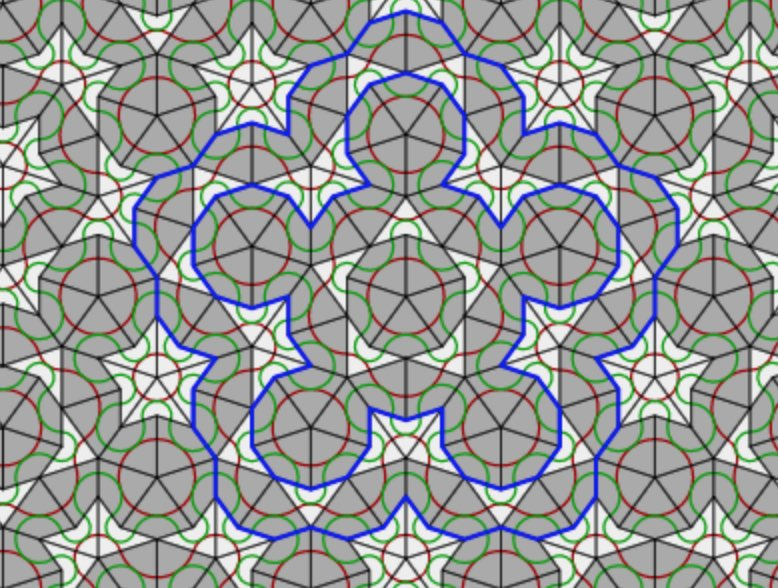The symmetry group of the standard $n$-dimensional hypercubic lattice
$\mathbb{Z} \vec{e}_1 + \dots + \mathbb{Z} \vec{e}_n \subset \mathbb{R}^n$
is the hyperoctahedral group of all signed $n \times n$ permutation matrices
$B_n = C_2^n \rtimes S_n$
in which all $n$-permutations $S_n$ act on the group $C_2^n = \{ 1,-1 \}^n$ of all signs. The signed permutation $n \times n$ matrix corresponding to an element $(\vec{a},\pi) \in B_n$ is given by
$T_{ij} = T(\vec{a},\pi)_{ij} = a_j \delta_{i,\pi(j)}$
The represenation theory of $B_n$ was worked out in 1930 by the British mathematician and clergyman Alfred YoungWe want to do explicit calculations in $B_n$ using a computational system such as GAP, so it is best to describe $B_n$ as a permutation subgroup of $S_{2n}$ via the morphism
$\tau((\vec{a},\pi))(k) = \begin{cases} \pi(k)+n \delta_{-1,a_k}~\text{if 1 \leq k \leq n} \\ \pi(k-n)+n(1-\delta_{-1,a_{k-n}})~\text{if n+1 \leq k \leq 2n} \end{cases}$
the image is generated by the permutations
$\begin{cases} \alpha = (1,2)(n+1,n+2), \\ \beta=(1,2,\dots,n)(n+1,n+2,\dots,2n), \\ \gamma=(n,2n) \end{cases}$
and to a permutation $\sigma \in \tau(B_n) \subset S_{2n}$ we assign the signed permutation $n \times n$ matrix $T_{\sigma}=T(\tau^{-1}(\pi))$.

We use GAP to set up $B_5$ from these generators and determine all its conjugacy classes of subgroups. It turns out that $B_5$ has no less than $953$ different conjugacy classes of subgroups.

gap> B5:=Group((1,2)(6,7),(1,2,3,4,5)(6,7,8,9,10),(5,10));
Group([ (1,2)(6,7), (1,2,3,4,5)(6,7,8,9,10), (5,10) ])
gap> Size(B5);
3840
gap> C:=ConjugacyClassesSubgroups(B5);;
gap> Length(C);
953

But we are only interested in the subgroups isomorphic to $D_5$. So, first we make a sublist of all conjugacy classes of subgroups of order $10$, and then we go through this list one-by-one and look for an explicit isomorphism between $D_5 = \langle x,y~|~x^5=e=y^2,~xyx=y \rangle$ and a representative of the class (or get a ‘fail’ is this subgroup is not isomorphic to $D_5$).

gap> C10:=Filtered(C,x->Size(Representative(x))=10);;
gap> Length(C10);
3
gap> s10:=List(C10,Representative);
[ Group([ (2,5)(3,4)(7,10)(8,9), (1,5,4,3,2)(6,10,9,8,7) ]),
Group([ (1,6)(2,5)(3,4)(7,10)(8,9), (1,10,9,3,2)(4,8,7,6,5) ]),
Group([ (1,6)(2,7)(3,8)(4,9)(5,10), (1,2,8,4,10)(3,9,5,6,7) ]) ]
gap> D:=DihedralGroup(10); gap> IsomorphismGroups(D,s10);
[ f1, f2 ] -> [ (2,5)(3,4)(7,10)(8,9), (1,5,4,3,2)(6,10,9,8,7) ]
gap> IsomorphismGroups(D,s10);
[ f1, f2 ] -> [ (1,6)(2,5)(3,4)(7,10)(8,9), (1,10,9,3,2)(4,8,7,6,5) ]
gap> IsomorphismGroups(D,s10);
fail
gap> IsCyclic(s10);
true

Of the three (conjugacy classes of) subgroups of order $10$, two are isomorphic to $D_5$, and the third one to $C_{10}$. Next, we have to transform the generating permutations into signed $5 \times 5$ permutation matrices using the bijection $\tau^{-1}$.
$\begin{array}{c|c} \sigma & (\vec{a},\pi) \\ \hline (2,5)(3,4)(7,10)(8,9) & ((1,1,1,1,1),(2,5)(3,4)) \\ (1,5,4,3,2)(6,10,9,8,7) & ((1,1,1,1,1)(1,5,4,3,2)) \\ (1,6)(2,5)(3,4)(7,10)(8,9) & ((-1,1,1,1,1),(2,5)(3,4)) \\ (1,10,9,3,2)(4,8,7,6,5) & ((-1,1,1,-1,1),(1,5,4,3,2)) \end{array}$
giving the signed permutation matrices
$\begin{array}{c|cc} & x & y \\ \hline A & \begin{bmatrix} 0 & 1 & 0 & 0 & 0 \\ 0 & 0 & 1 & 0 & 0 \\ 0 & 0 & 0 & 1 & 0 \\ 0 & 0 & 0 & 0 & 1 \\ 1 & 0 & 0 & 0 & 0 \end{bmatrix} & \begin{bmatrix} 1 & 0 & 0 & 0 & 0 \\ 0 & 0 & 0 & 0 & 1 \\ 0 & 0 & 0 & 1 & 0 \\ 0 & 0 & 1 & 0 & 0 \\ 0 & 1 & 0 & 0 & 0 \end{bmatrix} \\ \hline B & \begin{bmatrix} 0 & 1 & 0 & 0 & 0 \\ 0 & 0 & 1 & 0 & 0 \\ 0 & 0 & 0 & -1 & 0 \\ 0 & 0 & 0 & 0 & 1 \\ -1 & 0 & 0 & 0 & 0 \end{bmatrix} & \begin{bmatrix} -1 & 0 & 0 & 0 & 0 \\ 0 & 0 & 0 & 0 & 1 \\ 0 & 0 & 0 & 1 & 0 \\ 0 & 0 & 1 & 0 & 0 \\ 0 & 1 & 0 & 0 & 0 \end{bmatrix} \end{array}$
$D_5$ has $4$ conjugacy classes with representatives $e,y,x$ and $x^2$. the
character table of $D_5$ is
$\begin{array}{c|cccc} & (1) & (2) & (2) & (5) \\ & 1_a & 5_1 & 5_2 & 2_a \\ D_5 & e & x & x^2 & y \\ \hline T & 1 & 1 & 1 & 1 \\ V & 1 & 1 & 1 & -1 \\ W_1 & 2 & \tfrac{-1+ \sqrt{5}}{2} & \tfrac{-1 -\sqrt{5}}{2} & 0 \\ W_2 & 2 & \tfrac{-1 -\sqrt{5}}{2} & \tfrac{-1+\sqrt{5}}{2} & 0 \end{array}$
Using the signed permutation matrices it is easy to determine the characters of the $5$-dimensional representations $A$ and $B$
$\begin{array}{c|cccc} D_5 & e & x & x^2 & y \\ \hline A & 5 & 0 & 0 & 1 \\ B & 5 & 0 & 0 & -1 \end{array}$
decomosing into $D_5$-irreducibles as
$A \simeq T \oplus W_1 \oplus W_2 \quad \text{and} \quad B \simeq V \oplus W_1 \oplus W_2$
Representation $A$ realises $D_5$ as a rotation symmetry group of the hypercube lattice $\mathbb{Z}^5$ in $\mathbb{R}^5$, and next we have to find a $D_5$-projection $\mathbb{R}^5=A \rightarrow W_1 = \mathbb{R}^2$.

As a complex representation $A \downarrow_{C_5}$ decomposes as a direct sum of $1$-dimensional representations
$A \downarrow_{C_5} = V_1 \oplus V_{\zeta} \oplus V_{\zeta^2} \oplus V_{\zeta^3} \oplus V_{\zeta^4}$
where $\zeta = e^{2 \pi i /5}$ and where the action of $x$ on $V_{\zeta^i}=\mathbb{C} v_i$ is given by $x.v_i = \zeta^i v_i$. The $x$-eigenvectors in $\mathbb{C}^5$ are
$\begin{cases} v_0 = (1,1,1,1,1) \\ v_1 = (1,\zeta,\zeta^2,\zeta^3,\zeta^4) \\ v_2 =(1,\zeta^2,\zeta^4,\zeta,\zeta^3) \\ v_3 = (1,\zeta^3,\zeta,\zeta^4,\zeta^2) \\ v_4 = (1,\zeta^4,\zeta^3,\zeta^2,\zeta) \end{cases}$
The action of $y$ on these vectors is given by $y.v_i = v_{5-i}$ because
$x.(y.v_i) = (xy).v_i=(yx^{-1}).v_i=y.(x^{-1}.v_i) = y.(\zeta^{-i} v_i) = \zeta^{-1} (y.v_i)$
and therefore $y.v_i$ is an $x$-eigenvector with eigenvalue $\zeta^{5-i}$. As a complex $D_5$-representation, the factors of $A$ are therefore
$T = \mathbb{C} v_0, \quad W_1 = \mathbb{C} v_1 + \mathbb{C} v_4, \quad \text{and} \quad W_2 = \mathbb{C} v_2 + \mathbb{C} v_3$
But we want to consider $A$ as a real representation. As $\zeta^j = cos(\tfrac{2 \pi j}{5})+i~sin(\tfrac{2 \pi j}{5}) = c_j + i s_j$ hebben we can take the vectors in $\mathbb{R}^5$
$\begin{cases} \frac{1}{2}(v_1+v_4) = (1,c_1,c_2,c_3,c_4)= u_1 \\ -\frac{1}{2}i(v_1-v_4) = (0,s_1,s_2,s_3,s_4) = u_2 \\ \frac{1}{2}(v_2+v_3) = (1,c_2,c_4,c1,c3)= w_1 \\ -\frac{1}{2}i(v_2-v_3) = (0,s_2,s_4,s_1,s_3)= w_2 \end{cases}$
and $A$ decomposes as a real $D_5$-representation with
$T = \mathbb{R} v_0, \quad W_1 = \mathbb{R} u_1 + \mathbb{R} u_2, \quad \text{and} \quad W_2 = \mathbb{R} w_1 + \mathbb{R} w_2$
and if we identify $\mathbb{C}$ with $\mathbb{R}^2$ via $z \leftrightarrow (Re(z),Im(z))$ we can describe the $D_5$-projection morphism $\pi_{W_1}~:~\mathbb{R}^5=A \rightarrow W_1=\mathbb{R}^2$ via
$(y_0,y_1,y_2,y_3,y_4) \mapsto y_0+y_1 \zeta + y_2 \zeta^2 + y_3 \zeta^3 + y_4 \zeta^4 = \sum_{i=0}^4 y_i (c_i,s_i)$
Note also that $W_1$ is the orthogonal complement of $T \oplus W_2$, so is equal to the linear subspace in $\mathbb{R}^5$ determined by the three linear equations
$\begin{cases} \sum_{i=0}^4 x_i = 0 \\ \sum_{i=0}^4 c_{2i} x_i = 0 \\ \sum_{i=0}^4 s_{2i} x_i = 0 \end{cases}$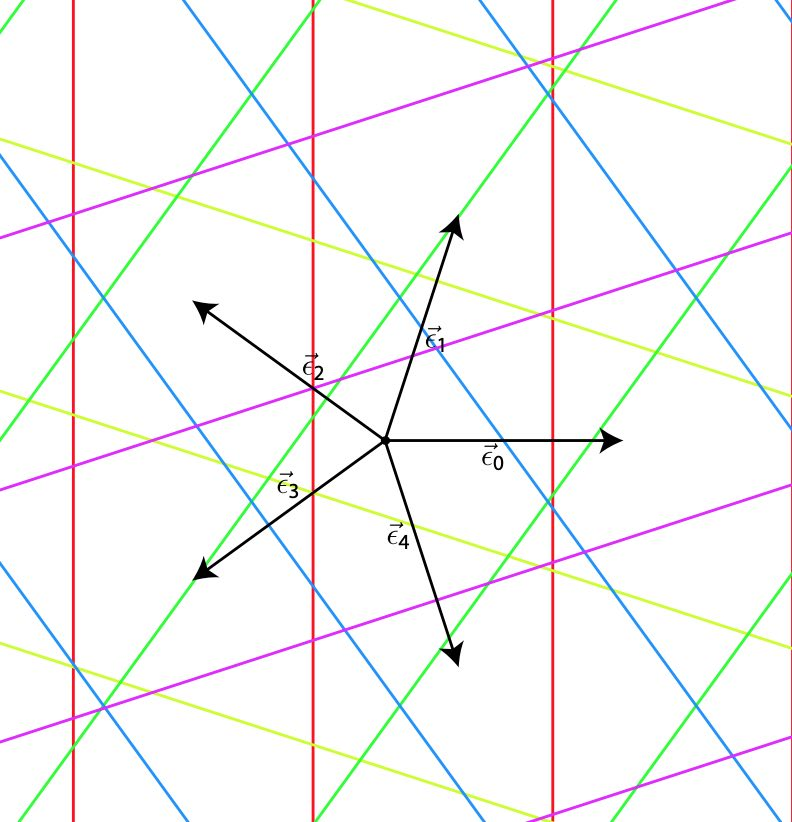Okay, now take the Rhombic tiling corresponding to the regular pentagrid defined by $\gamma_0, \dots, \gamma_4$ satisfying $\sum_{i=0}^4 \gamma_i = 0$. Let $\vec{k}=(k_0,\dots,k_4) \in \mathbb{Z}^5$ and define the open hypercube $H_{\vec{k}}$ corresponding to $\vec{k}$ as the set of points
$(x_0,\dots,x_4) \in \mathbb{R}^5~:~\forall 0 \leq i \leq 4~:~k_i – 1 < x_i < k_i$ From the vector $\vec{\gamma} = (\gamma_0,\dots,\gamma_4)$ determining the Rhombic tiling we define the $2$-dimensional plane $P_{\vec{\gamma}}$ in $\mathbb{R}^5$ given by the equations $\begin{cases} \sum_{i=0}^4 x_i = 0 \\ \sum_{i=0}^4 c_{2i} (x_i - \gamma_i) = 0 \\ \sum_{i=0}^4 s_{2i} (x_i - \gamma_i) = 0 \end{cases}$ The point being that $P_{\vec{\gamma}}$ is the linear plane $W_1$ in $\mathbb{R}^5$ translated over the vector $\vec{\gamma}$, so it is parallel to $W_1$. Here's the punchline:

de Bruijn’s theorem: The vertices of the Rhombic tiling produced by the regular pentagrid with parameters $\vec{\gamma}=(\gamma_0,\dots,\gamma_4)$ are the points
$\sum_{i=0}^4 k_i (c_i,s_i)$
with $\vec{k}=(k_0,\dots,k_4) \in \mathbb{Z}^5$ such that $H_{\vec{k}} \cap P_{\vec{\gamma}} \not= \emptyset$.

To see this, let $\vec{x} = (x_0,\dots,x_4) \in P_{\vec{\gamma}}$, then $\vec{x}-\vec{\gamma} \in W_1$, but then there is a vector $\vec{y} \in \mathbb{R}^2$ such that
$x_j – \gamma_j = \vec{y}.\vec{v}_j \quad \forall~0 \leq j \leq 4$
But then, with $k_j=\lceil \vec{y}.\vec{v}_j + \gamma_j \rceil$ we have that $\vec{x} \in H_{\vec{k}}$ and we note that $V(\vec{y}) = \sum_{i=0}^4 k_i \vec{v}_i$ is a vetex of the Rhombic tiling associated to the regular pentagrid parameters $\vec{\gamma}=(\gamma_0,\dots,\gamma_4)$.

Here we used regularity of the pentagrid in order to have that $k_j=\vec{y}.\vec{v}_j + \gamma_j$ can happen for at most two $j$’s, so we can manage to vary $\vec{y}$ a little in order to have $\vec{x}$ in the open hypercube.

Here’s what we did so far: we have identified $D_5$ as a group of rotations in $\mathbb{R}^5$, preserving the hypercube-lattice $\mathbb{Z}^5$ in $\mathbb{R}^5$. If the $2$-plane $P_{\vec{\gamma}}$ is left stable under these rotations, then because rotations preserve distances, also the subset of lattice-points
$S_{\vec{\gamma}} = \{ (k_0,\dots,k_4)~|~H_{\vec{k}} \cap P_{\vec{\gamma}} \not= \emptyset \} \subset \mathbb{Z}^5$
is left stable under the $D_5$-action. But, because the map
$(k_0,\dots,k_4) \mapsto \sum_{i=0}^4 k_i (c_i,s_i)$
is the $D_5$-projection map $\pi : A \rightarrow W_1$, the vertices of the associated Rhombic tiling must be stable under the $D_5$-action on $W_1$, meaning that the Rhombic tiling should have a global $D_5$-symmetry.

Sadly, the only plane $P_{\vec{\gamma}}$ left stable under all rotations of $D_5$ is when $\vec{\gamma} = \vec{0}$, which is an exceptionally singular pentagrid. If we project this situation we do indeed get an image with global $D_5$-symmetry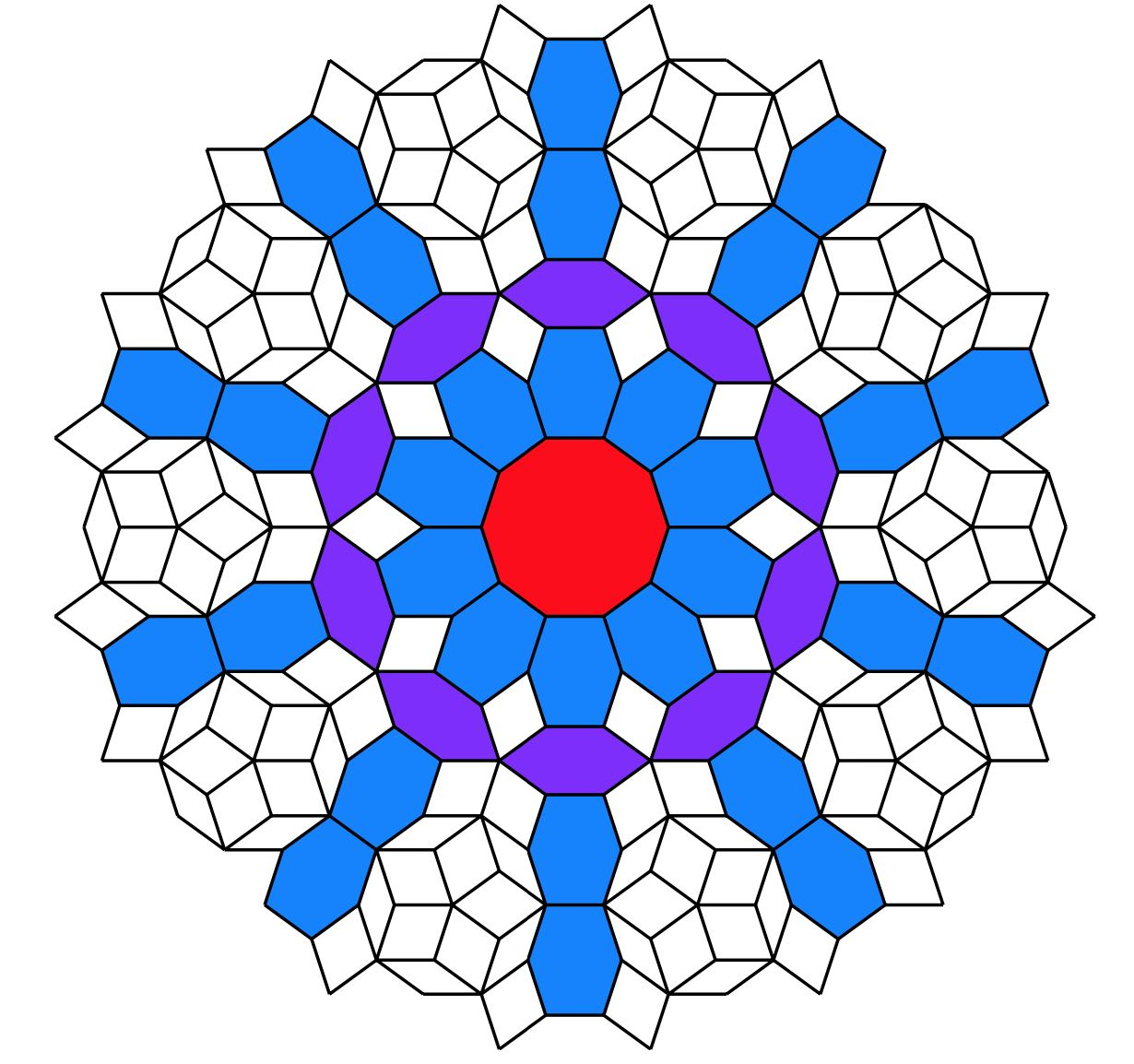but it is not a Rhombic tiling. What’s going on?

Because this post is already dragging on for far too long (TL;DR), we’ll save the investigation of projections of singular pentagrids, how they differ from the regular situation, and how they determine multiple Rhombic tilings, for another time.

In a Rhombic tiling (aka a Penrose P3 tiling) we can identify five ribbons.

Opposite sides of a rhomb are parallel. We may form a ribbon by attaching rhombs along opposite sides. There are five directions taken by sides, so there are five families of ribbons that do not intersect, determined by the side directions.

Every ribbon determines a skeleton curve through the midpoints of opposite sides of the rhombs. If we straighten these skeleton curves we get a de Bruijn’s pentagrid.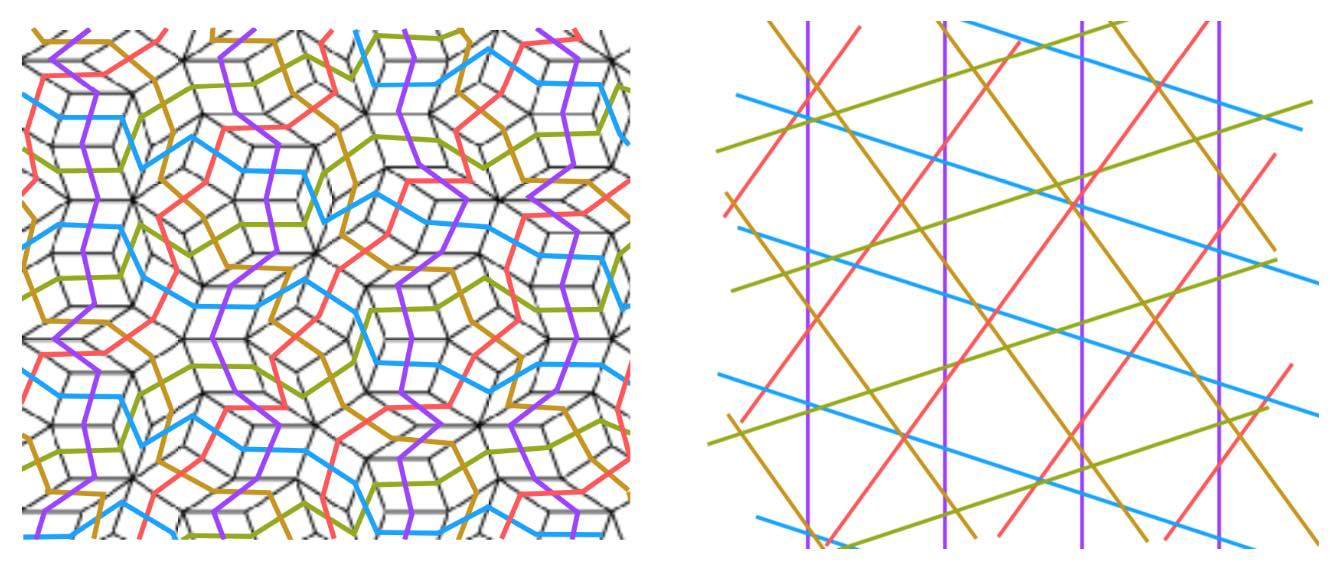A pentagrid is a grid of the plane consisting of five families of parallel lines, at angles multiples of $72^o = 360^o/5$, and with each family consisting of parallel lines with a spacing given by a number $\gamma_i$ for $0 \leq i \leq 4$, satisfying
$\gamma_0+\gamma_1+\gamma_2+\gamma_3+\gamma_4=0$

The points of the plane in the $j$-th family of the pentagrid are
$\{ \vec{x} \in \mathbb{R}^2~|~\vec{x}.\vec{v}_j + \gamma_j \in \mathbb{Z} \}$
where $\vec{v}_j = \zeta^j = (cos(2 \pi j/5),sin(2 \pi j/5))$.

A pentagrid is regular if no point in $\mathbb{R}^2$ belongs to more than two of the five grids. For almost all choices $\gamma_0,\dots,\gamma_4$ the corresponding pentagrid is regular. The pentagrid coordinates of a point $\vec{x} \in \mathbb{R}^2$ are the five integers $(K_0(\vec{x}),\dots,K_4(\vec{x}) \in \mathbb{Z}^5$ defined by
$K_j(\vec{x}) = \lceil \vec{x}.\vec{v}_j + \gamma_j \rceil$
with $\lceil r \rceil$ the smallest integer greater of equal to $r$, and clearly the function $K_j(\vec{x})$ is constant in regions between the $j$-th grid lines.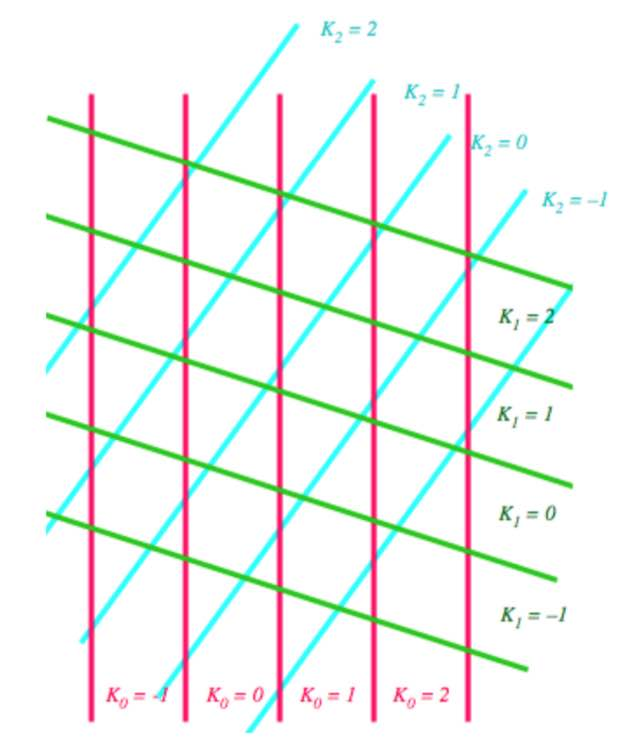With these pentagrid-coordinates one can associate a vertex to any point $\vec{x} \in \mathbb{R}^2$
$V(\vec{x}) = \sum_{j=0}^4 K_j(\vec{x}) \vec{v}_j$
which is constant in regions between grid lines.

Here’s de Bruijn‘s result:

For $\vec{x}$ running over $\mathbb{R}^2$, the vertices $V(\vec{x})$ coming from a pentagrid determine a Rhombic tiling of the plane. Even better, every Rhombic tiling comes from a pentagrid.We’ll prove this for regular pentagrids (the singular case follows from a small deformation).

Any intersection point $\vec{x}_0$ of the pentagrid belongs to exactly two families of parallel lines, so we have two integers $r$ and $s$ with $0 \leq r < s \leq 4$ and integers $k_r,k_s \in \mathbb{Z}$ such that $\vec{x}_0$ is determined by the equations $\begin{cases} \vec{x}.\vec{v}_r + \gamma_r = k_r \\ \vec{x}.\vec{v}_s + \gamma_s = k_s \end{cases}$ In a small neighborhood of $\vec{x}_0$, $V(\vec{x})$ takes the values of four vertices of a rhomb. These vertices are associated to the $5$-tuples in $\mathbb{Z}^5$ given by $(K_0(\vec{x}_0),\dots,K_4(\vec{x}_0))+\epsilon_1 (\delta_{0r},\dots,\delta_{4r})+\epsilon_2 (\delta_{0s},\dots,\delta_{4s})$ with $\epsilon_1,\epsilon_2 \in \{ 0,1 \}$. So, the intersection points of the regular pentagrid lines correspond to rhombs, and the regions between the grid lines (which are called meshes) correspond to vertices, whose positions are given by $V(\vec{x})$.

Observe that these four vertices give a thin rhombus if the angle between $\vec{v}_r$ and $\vec{v}_s$ is $144^o$ and determine a thick rhombus if the angle is $72^o$.

Not all pentagrid coordinates $(k_0,\dots,k_4)$ occur in the tiling though. For $\vec{x} \in \mathbb{R}^2$ we have
$K_j(\vec{x}) = \vec{x}.\vec{v}_j + \gamma_j + \lambda_j(\vec{x})$
with $0 \leq \lambda_j(\vec{x}) < 1$. In a regular pentagrid at most two of the $\lambda_j(\vec{x})$ can be zero and so we have $0 < \lambda_0(\vec{x}) + \dots + \lambda_4(\vec{x}) < 5$ and we have assumed that $\gamma_0 + \dots + \gamma_4 = 0$, giving us $\sum_{j=0}^4 K_j(\vec{x}) = \vec{x}.(\sum_{j=0}^4 \vec{v}_j) + \sum_{j=0}^4 \gamma_j + \sum_{j=0}^4 \lambda_j(\vec{x}) = \sum_{j=0}^4 \lambda_j(\vec{x})$ The left hand side must be an integers and the right hand side a number strictly between $0$ and $5$. This defines the index of a vertex
$ind(\vec{x}) = \sum_{j=0}^4 K_j(\vec{x}) \in \{ 1,2,3,4 \}$
Therefore, every vertex in the tiling may be represented as
$k_0 \vec{v}_0 + \dots + k_4 \vec{v}_4$
with $(k_0,\dots,k_4) \in \mathbb{Z}^5$ satisfying $\sum_{j=0}^4 k_j \in \{ 1,2,3,4 \}$. If we move a point along the edges of a rhombus, we note that the index increases by $1$ in the directions of $\vec{v}_0,\dots,\vec{v}_4$ and decreases by $1$ in the directions $-\vec{v}_0,\dots,-\vec{v}_4$.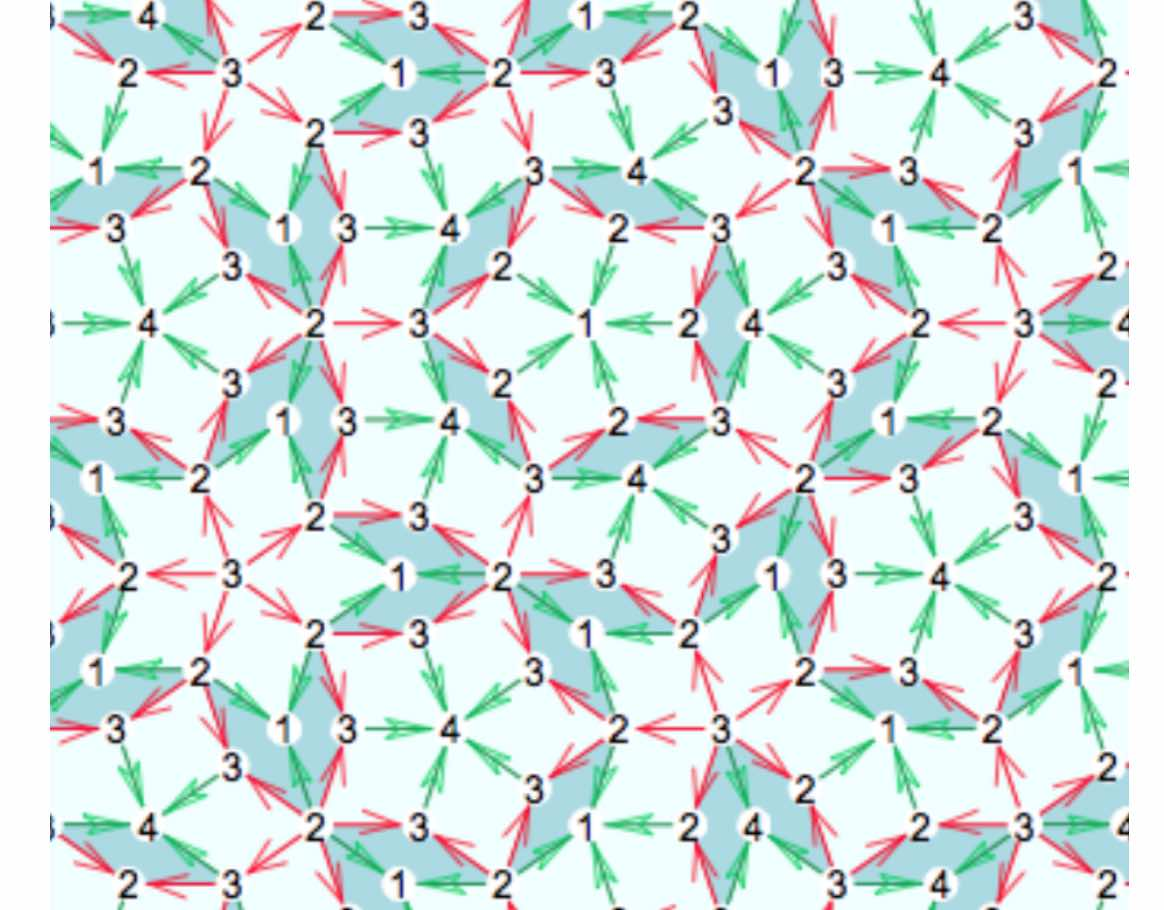It follows that the index-values of the vertices of a thick rhombus are either $1$ and $3$ at the $72^o$ angles and $2$ at the $108^o$ angles, or they are $2$ and $4$ at the $72^o$ angles, and $3$ at the $108^o$ angles. For a thin rhombus the index-values must be either $1$ and $3$ at the $144^o$ angles and $2$ at the $36^o$ angles, or $2$ and $4$ at the $144^o$ angles and $3$ at the $36^o$ angles.

We still have to show that this gives a legal Rhombic tiling, that is, that the gluing restrictions of Penrose’s rhombs are satisfied.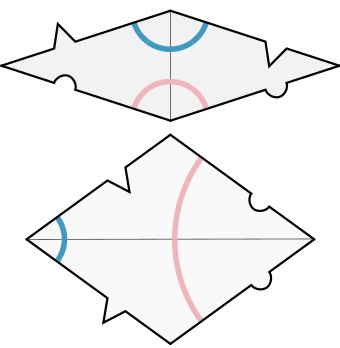We do this by orienting and colouring the edges of the thin and thick rhombus towards the vertex defined by the semi-circles on the rhombus-pieces. Edges connecting a point of index $3$ to a point of index $2$ are coloured red, edges connecting a point of index $1$ to a point of index $2$, or connecting a point of index $3$ to one of index $4$ are coloured blue.

We orient green edges pointing from index $2$ to index $1$, or from index $3$ to index $4$. As this orientation depends only on the index-values of the vertices, two rhombs sharing a common green edge also have the same orientation on that edge.

On each individual rhomb, knowing the green edges and their orientation forced the red edges and their orientation, but we still have to show that if two rhombs share a common red edge, this edge has the same orientation on both (note in the picture above that a red edge can both flow from $2$ to $3$ as well as from $3$ to $2$).

From the gluing conditions of Penrose’s rhombs we see that if $PQ$ be a red edge, in common to two rhombs, and these rhombs have angle $\alpha$ resp. $\beta$ in $P$, then $\alpha$ and $\beta$ must be both less that $90^o$ or both bigger than $90^o$. We translate this in terms of the pentragrid.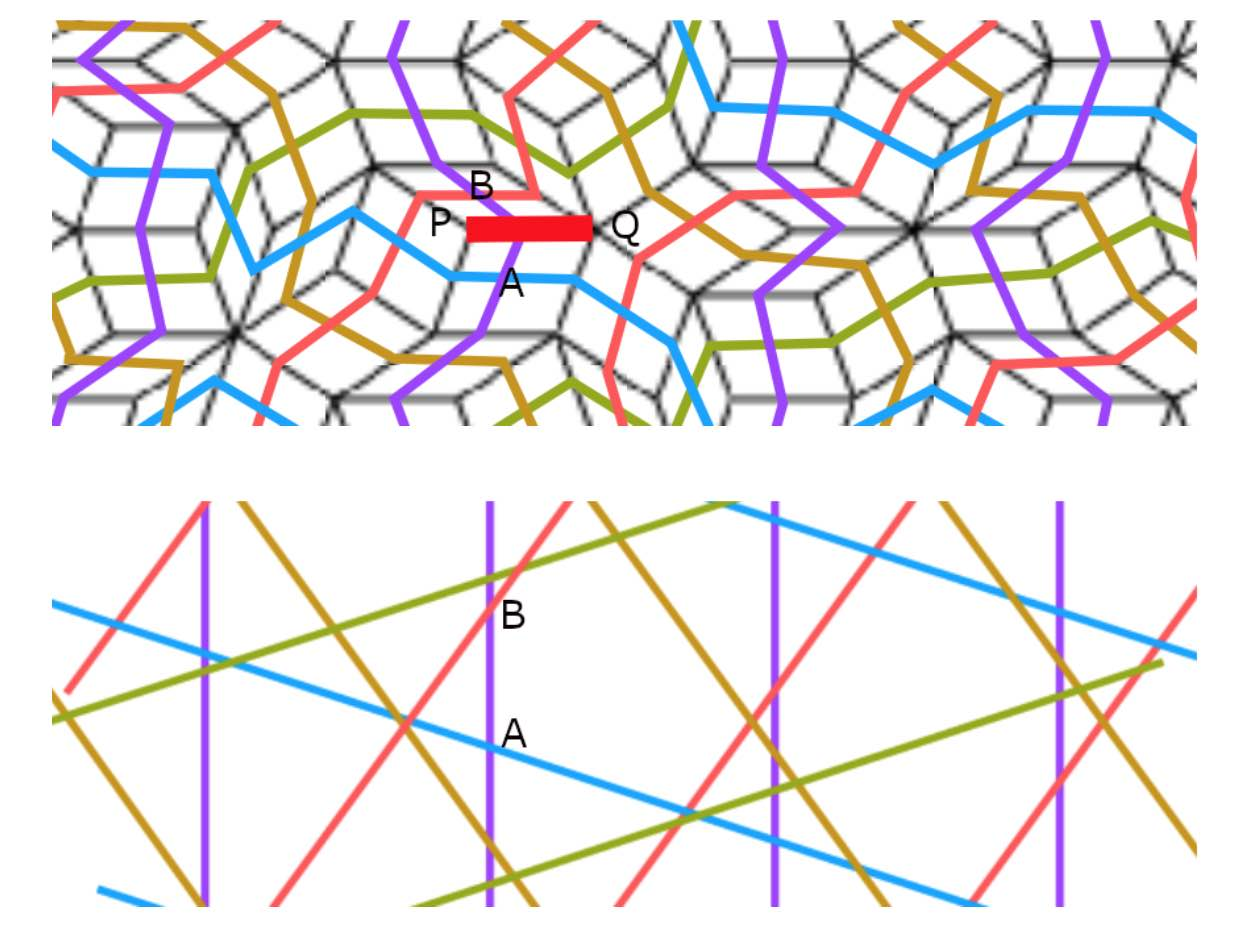The two rhombs correspond to two intersection points $A$ and $B$, and we may assume that they both lie on a line $l$ of family $0$ of parallel lines (other cases are treated similarly by cyclic permutation), and that $A$ is an intersection with a family $p$-line and $B$ with a family $q$-line, with $p,q \in \{ 1,2,3,4 \}$. The interval $AB$ crosses the unique edge common to the two rhombs determined by $A$ and $B$, and we call the interval $AB$ red if $\sum_j K_j(\vec{x})$ is $2$ on one side of $AB$ and $3$ on the other side. The claim about the angles $\alpha$ and $\beta$ above now translates to: if $AB$ is red, then $p+q$ is odd (in the picture above $p=1$ and $q=2$).

By a transformation we may assume that $\gamma_0=0$ and that $l$ is the $Y$-axis. For every $y \in \mathbb{R}$ we then get
$\begin{cases} K_1(0,y) = \lceil y .sin(2 \pi/5) + \gamma_1 \rceil \\ K_2(0,y) = \lceil y .sin(4 \pi/5) + \gamma_2 \rceil \\ K_3(0,y) = \lceil -y .sin(4 \pi/5) + \gamma_3 \rceil \\ K_4(0,y) = \lceil -y .sin(2 \pi/5) + \gamma_4 \rceil \end{cases}$
and $\gamma_1+\gamma_4$ and $\gamma_2+\gamma_3$ are not integers (otherwise the pentagrid is not regular). If $y$ runs from $-\infty$ to $+\infty$ we find that
$K_1(0,y)+K_4(0,y) – \lceil \gamma_1 + \gamma_4 \rceil = \begin{cases} 0 \\ 1 \end{cases}$
with jumps from $0$ to $1$ at places where $(\lceil \gamma_1 + \gamma_4 \rceil – \gamma_1)/sin(2 \pi/5) \in \mathbb{Z}$ and from $1$ to $0$ when $\gamma_4/sin(2 \pi/5) \in \mathbb{Z}$. (A similar result holds replacing $K_1,K_4,\gamma_1,\gamma_4,sin(2 \pi/5)$ by $K_2,K_3,\gamma_2,\gamma_3,sin(4 \pi/5)$.

Because the points on $l$ intersecting with $1$-family and $4$-family gridlines alternate (and similarly for $2$- and $3$-family gridlines) we know already that $p \not= q$. If we assume that $p+q$ is even, we have two possibilities, either $\{p,g \}=\{ 1,3 \}$ or $\{ 2,4 \}$. As $\gamma_0=0$ we have $\gamma_1+\gamma_2+\gamma_3+\gamma_4=0$ and therefore
$\lceil \gamma_1 + \gamma_4 \rceil + \lceil \gamma_2+\gamma_3 \rceil = 1$
It then follows that $K_1(0,y)+K_2(0,y)+K_3(0,y)+K_4(0,y) = 1$ or $3$ between the points $A$ and $B$. Therefore $K_0(y,0) + \dots + K_4(0,y)$ is either $1$ on the left side and $2$ on the right side, or is $3$ on the left side and $4$ on the right side, so $AB$ must be green, a contradiction. Therefore, $p+q$ is odd, and the orientation of the red edge in both rhombs is the same. Done!

An alternative way to see this correspondence between regular pentagrids and Rhombic tilings as as follows. To every intersection of two gridlines we assign a rhombus, a thin one if they meet at an acute angle of $36^o$ and a thich one if this angle is $72^o$, with the long diagonal dissecting the obtuse angle.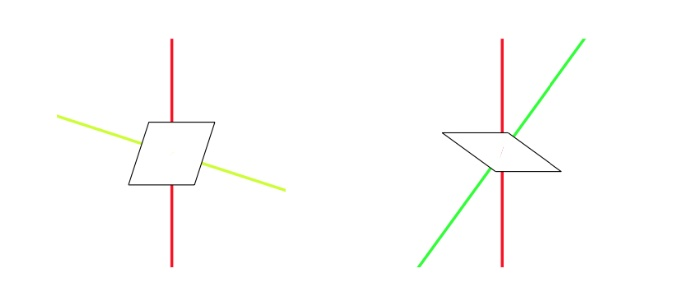Do this for all intersections, surrounding a given mesh.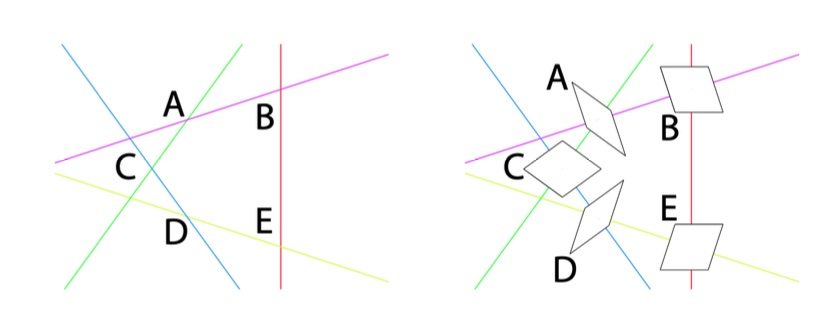Attach the rhombs by translating them towards the mesh, and finally draw the colours of the edges.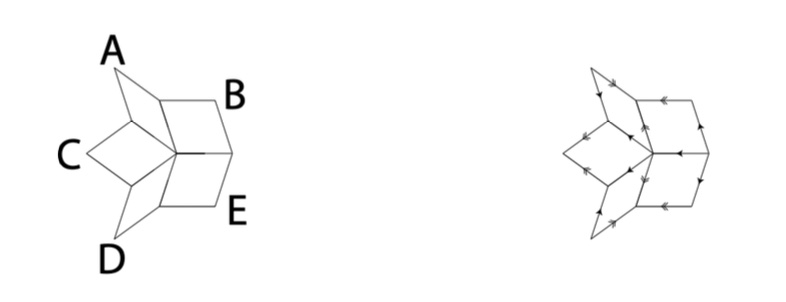Another time, we will connect this to the cut-and-project method, using the representation theory of $D_5$.

Around 1975 Sir Roger Penrose discovered his aperiodic P2 tilings of the plane, using only two puzzle pieces: Kites (K) and Darts (D)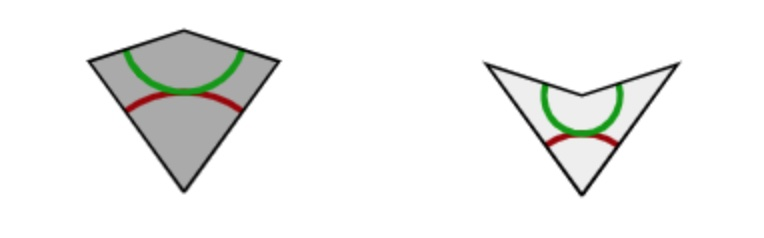The inner angles of these pieces are all multiples of $36^o = \tfrac{180^o}{5}$, the short edges have length $1$, and the long edges have length $\tau = \tfrac{1+\sqrt{5}}{2}$, the golden ratio. These pieces must be joined together so that the colours match up (if we do not use this rule it is easy to get periodic tilings with these two pieces).

There is plenty of excellent online material available:

• The two original Martin Gardner Scientific American articles on Penrose tiles have been made available by the MAA, and were reprinted in “Penrose Tiles to Trapdoor Ciphers”. They contain most of Conway’s early discoveries about these tilings, but without proofs.
• A JavaScript application by Kevin Bertman to play around with these tilings. You can deflate and inflate tilings, find forced tiles and much more. Beneath the app-window there’s a detailed explanation of all the basics, including inflation and deflation of the P2-tiles, the seven types of local vertex configurations (naming by Conway, of course),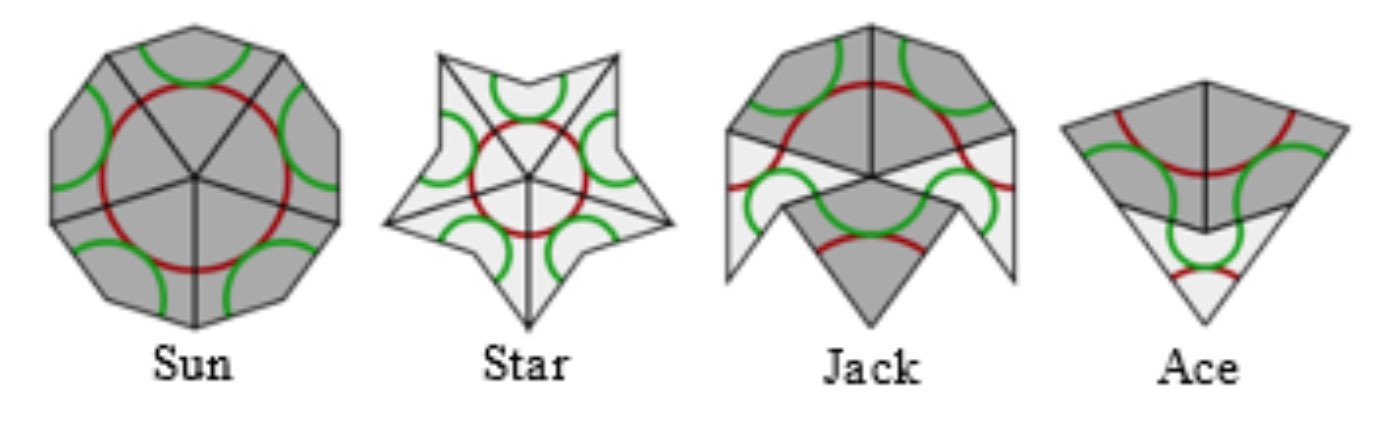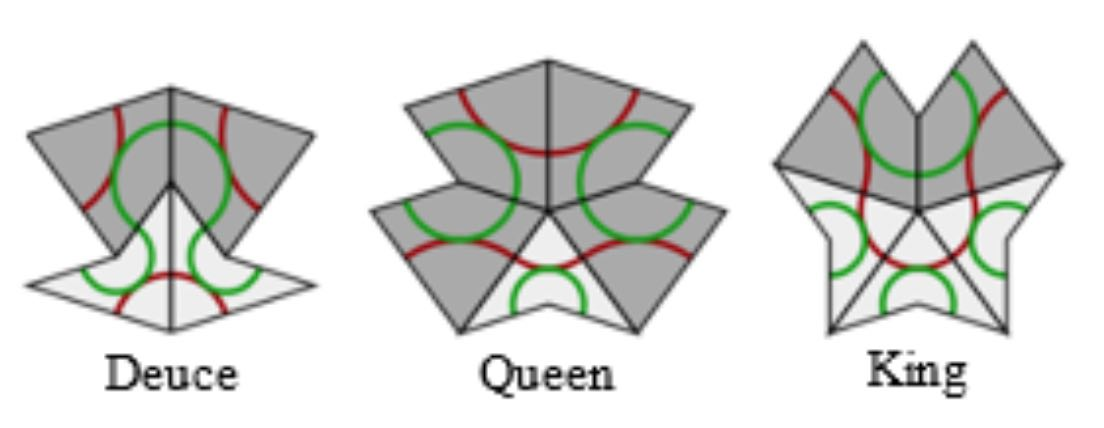proofs of aperiodicity (similar to the one for Conway’s musical sequences), that every tile lies within an ace (similar to the LSL-subword in musical sequences) with application to local isomorphism (again similar to the $1$-dimensional case).
• Course notes of an Oxford masterclass in geometry Lectures on Penrose tilings by Alexander Ritter, again with proofs of all of the above and a discussion about the Cartwheel tilings (similar to that in the post on musical sequences), giving an algorithm to decide whether or not a partial tiling can be extended to the entire plane, or not.

There’s no point copying this material here. Rather, I’d like to use some time in this GoV series of posts to talk about de Bruijn’s pentagrid results. For this reason, I now need to make the connection with Penrose’s ‘other’ tilings, the P3 tiles of ‘thin’ and ‘thick’ rhombi (sometimes called ‘skinny’ and ‘fat’ rhombi).Every Penrose P2-tiling can be turned into a P3-rhombic tiling, and conversely.

From kites and darts to rhombi: divide every kite in two halves along its line of reflection. Then combine darts and half-kites into rhombi where a fat rhombus consists of a dart and two half-kites, joined at a long edge, and a skinny rhombus is made of two half-kites, joined at a short edge. This can always be done preserving the gluing conditions. It suffices to verify this for the deflated kite and dart (on the left below) and we see that the matching colour-conditions are those of the rhombi.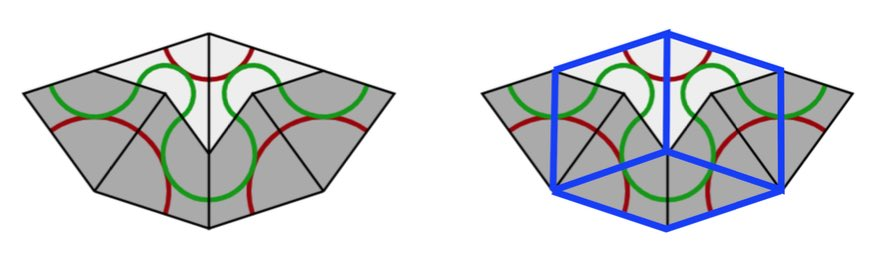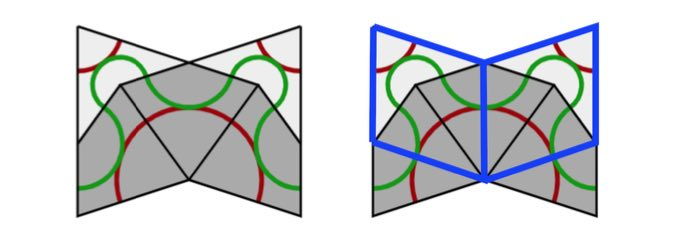From rhombi to kites and darts: divide every fat rhombus into a dart (placed at the red acute angle) and two half-kites, joined at a long edge, and divide every skinny rhombus into two half-kites along its short diagonal.

All results holding for Kites and Darts tilings have therefore their versions for Rhombic tilings. For example, we have rhombic deflation and inflation rules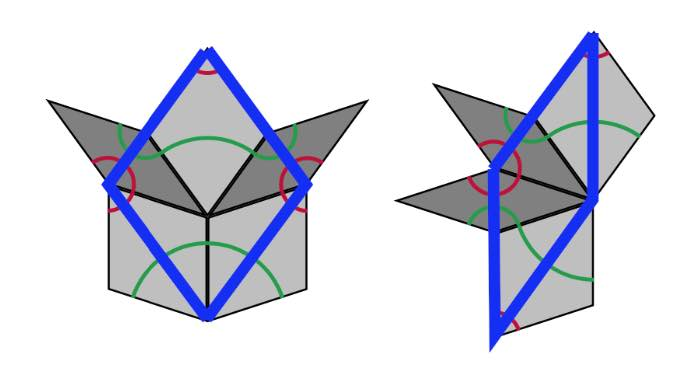A Rhombic tiling can be seen as an intermediate step in the inflation process of a Penrose tiling $P$. Start by dividing all kites in two halves along their long diagonal and all darts in two halves along their short diagonal (the purple lines below).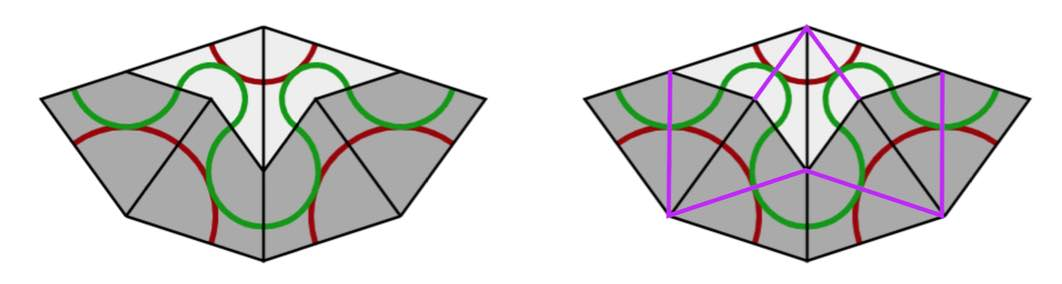If we consider all original black lines together with the new purple ones, we get a tiling of the plane by triangles and we call this the $A$-tiling. There are two triangles, s small triangle $S_A$ (the whiter ones, with two small edges ofd length $1$ and one edge of length $\tau$) and a large triangle $L_A$ (the greyer ones, with two edges of length $\tau$ and one edge of length $1$).

Next, we remove the black lines joining an $S_A$-triangle with an $L_A$-triangle, and get another tiling with triangles, the $B$-tiling, with two pieces, a large triangle $L_B$ with two sides of length $\tau$ and one side of length $1+\tau$ (the white ones) (the union of an $L_A$ and a $S_A$), and a small triangle $S_B$ which has the same form as $L_A$ (the greyer ones). If we now join two white triangles $L_B$ along their common longer edge, and two greyer triangles $S_B$ along their common smaller edge, we obtain the Rhombic tiling $R$ corresponding to $P$.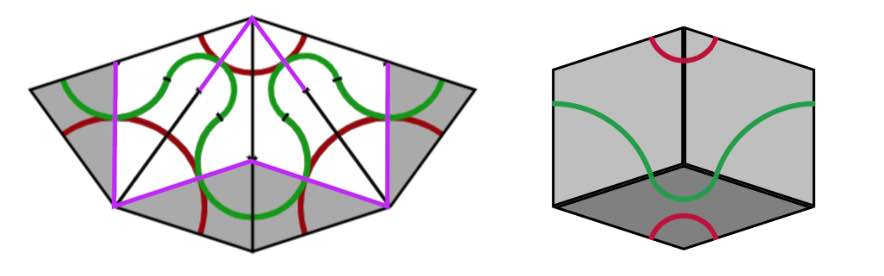We can repeat this process starting with the Rhombic tiling $R$. We divide all fat rhombi in two along their long diagonals, and the skinny rhombi in two along their short diagonals (the purple lines). We obtain a tiling of the plane by triangles, which is of course just the $B$-tiling above.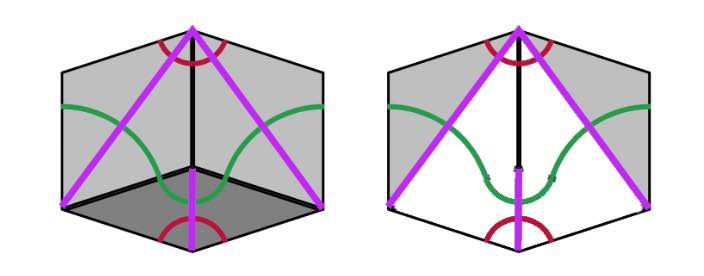Remove the edge joining a small $S_B$ with a large $L_B$ triangle, then we get a new tiling by triangles, the $\tau A$-tiling consisting of large triangles $L_{\tau A}$ (the white ones) with two long edges of length $1+\tau$ and one short edge of length $\tau$ (note that $L_{\tau A}$ is $\tau$ times the triangle $L_A$), and a smaller one $S_{\tau A}$ (the grey ones) having two short edges of length $\tau$ and one long edge of length $1+\tau$ (again $S_{\tau A}$ is $\tau$ times the triangle $S_A$). If we join two $L_{\tau A}$-triangles sharing a common long edge we obtain a Kite ($\tau$-times larger than the original Kite) and if we joint two $S_{\tau A}$-triangles along their common small edge we get a Dart ($\tau$ times larger than the original Dart). The Penrose tiling we obtain is the inflation $inf(P)$ of the original $P$.

If we repeat the whole procedure starting from $inf(P)$ instead of $P$ we get, in turn, triangle tilings of the plane, subsequently the $\tau B$-tiling, the $\tau^2 A$-tiling, the $\tau^2 B$-tiling and so on, the triangles in a $\tau^n A$-tiling being of size $\tau^2$-times those of the $A$-tiling, and those in the $\tau^n B$-tiling $\tau^n$-times as large as those of the $B$-tiling.

The upshot of this is that we can associate to a Penrose tiling $P$ of the plane a sequence of $0$’s and $1$’s. Starting from $P$ we have an infinite sequence of associated triangle tilings
$A,~B,~\tau A,~\tau B,~\tau^2 A, \dots,~\tau^n A,~\tau^n B,~\tau^{n+1} A, \dots$
with larger and larger triangle tiles. Let $p$ be a point of the plane lying in the interior of an $A$-tile, then we define its index sequence
$i(P,p) = (x_0,x_1,x_2,\dots ) \quad x_{2n} = \begin{cases} 0~\text{if p \in L_{\tau^n A}} \\ 1~\text{if p \in S_{\tau^n A}} \end{cases}~ x_{2n+1} = \begin{cases} 0~\text{if p \in L_{\tau^n B}} \\ 1~\text{if p \in S_{\tau^n B}} \end{cases}$
That is, $x_0=1$ if $p$ lies in a small triangle of the $A$-tiling and $x_0=1$ if it lies in a large triangle, $x_1=1$ if $p$ lies in a small triangle of the $B$-tiling and is $0$ if it lies in a large $B$-triangle, and so on.

The beauty of this is that every infinite sequence $(x_0,x_1,x_2,\dots )$ whose terms are $0$ or $1$, and in which no two consecutive terms are equal to $1$, is the index sequence $i(P,p)$ of some Penrose tiling $P$ in some point $p$ of the plane.

From the construction of the sequence of triangle-tilings it follows that a small triangle is part of a large triangle in the next tiling. For this reason an index sequence can never have two consecutive ones. An index sequence gives explicit instructions as to how the Penrose tiling is constructed. In each step add a large or small triangle as to fit the sequence, together with the matching triangle (the other half of a Penrose or Rhombic tile). Next, look at the patches $P_i$ of Kites and Darts (in the $\tau^i A$ tiling), for $i=0,1,\dots$, then $P_{i-k}$ is contained in the $k$-times inflated $P_i$, $i^k(P_i)$ (without rescaling), for each $i$ and all $1 \leq k \leq i$. But then, we have a concentric series of patches
$P_0 \subset i(P_1) \subset i^2(P_2) \subset \dots$
filling the entire plane.The index sequence depends on the choice of point $p$ in the plane. If $p’$ is another point, then as the triangle tiles increase is size at each step, $p$ and $p’$ will lie in the same triangle-tile at some stage, and after that moment their index sequences will be the same.

As a result, there exists an uncountable infinity of distinct Penrose tilings of the plane.

From the discussions we see that a Penrose tiling is determined by the index-sequence in any point in the plane $(x_0,x_1,\dots )$ consisting of $0$’s and $1$’s having no two consecutive $1$’s, and that another such sequence $(x’_0,x’_1,\dots )$ determines the same Penrose tilings if $x_n=x’_n$ for all $n \geq N$ for some number $N$. That is, the Penrose tiling is determined by the the equivalence class of such sequences, and it is easy to see that there are uncountably many such equivalence classes.

If you want to play a bit with Penrose tiles, you can order the P2 tiles or the P3 tiles from
Cherry Arbor Design.

A Conway musical sequence is an infinite word in $L$ and $S$, containing no two consecutive $S$’s nor three consecutive $L$’s, such that all its inflations remain musical sequences.

We’ve seen that such musical sequences encode an aperiodic tiling of the line in short ($S$) and long ($L$) intervals, and that such tilings are all finite locally isomorphic.

But, apart from the middle $C$-sequences (the one-dimensional cartwheel tilings) we gave no examples of such tilings (or musical sequences). Let’s remedy this!

Take any real number $c$ as long as it is not an integral combination of $1$ and $\tfrac{1}{\tau}$ (with $\tau$ the golden ratio) and assign to any integer $a \in \mathbb{Z}$ a tile
$P_c(a) = \begin{cases} S \\ L \end{cases} ~\text{iff}~\lceil c+(a+1)\frac{1}{\tau} \rceil – \lceil c+a \frac{1}{\tau} \rceil = \begin{cases} 0 \\ 1 \end{cases}$
(instead of ceilings we might have taken floors, because of the restriction on $c$).

With a little bit of work we see that the deflated word determined by $P_c$ is again of this type, more precisely $def(P_c) = P_{-(c-\lfloor c \rfloor)\frac{1}{\tau}}$. But then it also follows that inflated words are of this type, meaning that all $P_c$ define a musical sequence.

Let’s just check that these sequences satisfy the gluing restrictions. If there is no integer between $c+a\tfrac{1}{\tau}$ and $c+(a+1)\tfrac{1}{\tau}$, because $2 \tfrac{1}{\tau} \approx 1.236$ there must be an interval in the preceding and the following $\tfrac{1}{\tau}$-interval, showing that an $S$ in the sequence has an $L$ on its left and right, so there are no two consecutive $S$’s in the sequences.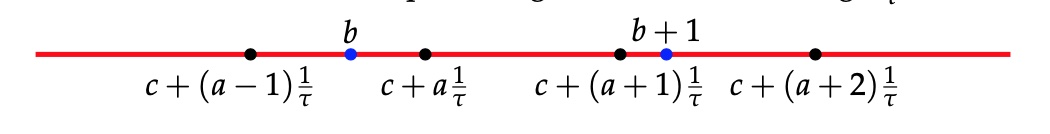Similarly, if two consecutive $\tfrac{1}{\tau}$-intervals have an integer in them, the next interval cannot contain an integer as $3 \tfrac{1}{\tau} \approx 1.854 < 2$.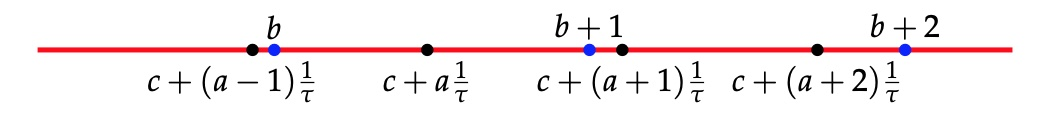Now we come to the essential point: these sequences can be obtained by the cut-and-project method.

Take the line $L$ through the origin with slope $\tfrac{1}{\tau}$ and $L^{\perp}$ the line perpendicular it.

Consider the unit square $H$ and $H_{\vec{\gamma}}=H + \vec{\gamma}$ its translation under a shift vector $\vec{\gamma}=(\gamma_x,\gamma_y)$ and let $\pi$ (or $\pi^{\perp}$) be the orthogonal projection of the plane onto $L$ (or onto $L^{\perp}$). One quickly computes that
$\pi(a,b) = (\frac{\tau^2 a + \tau b}{1+\tau^2},\frac{\tau a + b}{1+\tau^2}) \quad \text{and} \quad \pi^{\perp}(a,b) = (\frac{a-\tau b}{1+\tau^2},\frac{\tau^2b-\tau a}{1+\tau^2})$
In the picture, we take $\vec{\gamma}=(c,-\tau c)$.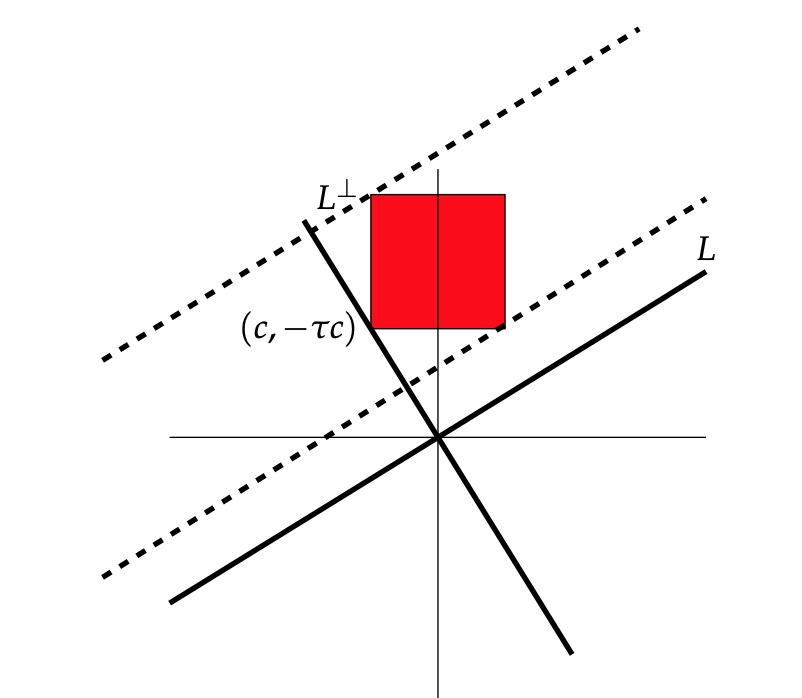The window $W$ will be the strip, parallel with $L$ with basis $\pi^{\perp}(H_{\vec{\gamma}})$.

We cut the standard lattice $\mathbb{Z}^2$, of all points with integer coordinates in the plane, by retricting to the window $\mathcal{P}=\mathbb{Z}^2 \cap W$.

Next, we project $\mathcal{P}$ onto the line $L$, and we get a set of endpoints of intervals which divide the line $L$ into short intervals of length $\tfrac{1}{\sqrt{1+\tau^2}}$ and long intervals of length $\tfrac{\tau}{\sqrt{1+\tau^2}}$.

For $(a,b) \in W$, the interval will be short if $(a,b+1) \in W$ and long if $(a+1,b) \in W$.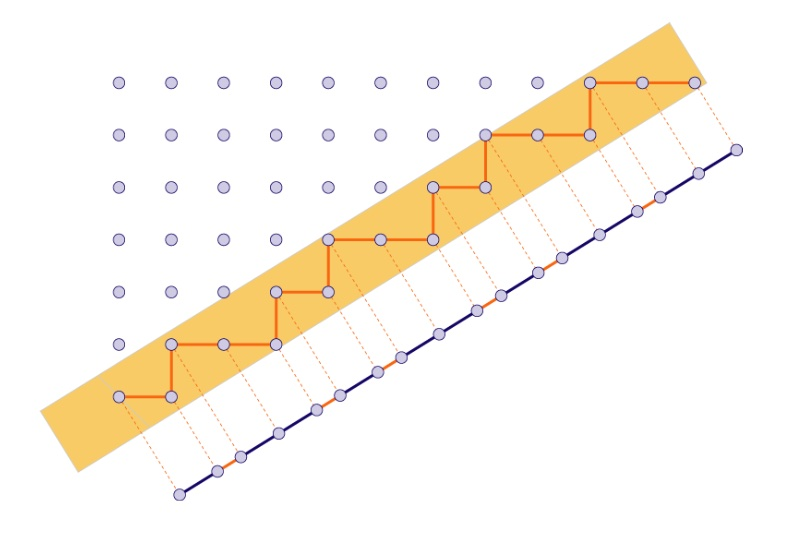Because these intervals differ by a factor $\tau$ in length, we get a tiling of the line by short intervals $S$ and long intervals $L$. It is easy to see that they satisfy the gluing restrictions (remember, no two consecutive short intervals and no three consecutive long intervals): the horizontal width of the window $W$ is $1+\tau \approx 2.618$ (so there cannot be three consecutive long intervals in the projection) and the vertical width of the window $W$ is $1+\tfrac{1}{\tau} = \tau \approx 1.618$ so there cannot be two consecutive short intervals in the projection.

Ready for the punchline?

The sequence obtained from projecting $\mathcal{P}$ is equal to the sequence $P_{(1+\tau^2)c}$. So, we get all musical sequences of this form from the cut-and-project method!

On $L^{\perp}$ the two end-points of the window are
$\begin{cases} \pi^{\perp}(c+1,-\tau c) = (\frac{(1+\tau^2)c+1}{1+\tau^2},- \tau \frac{(1+\tau^2)c+1}{1+\tau^2}) \\ \pi^{\perp}(c,-\tau c+1) = (\frac{(1+\tau^2)c-\tau}{1+\tau^2},-\tau \frac{(1+\tau^2)c-\tau}{1+\tau^2}) \end{cases}$
Therefore, a point $(a,b) \in \mathbb{Z}^2$ lies in the window $W$ if and only if
$(1+\tau^2)c-\tau < a-\tau b < (1+\tau^2)c+1$ or equivalently, if $(1+\tau^2)c+(b-1)\tau < a < (1+\tau^2)c+b \tau + 1$ Observe that $\lceil (1+\tau^2)c + b\tau \rceil - \lceil (1+\tau^2)c+(b-1)\tau \rceil = P_{(1+\tau^2)c}(b-1) + 1 \in \{ 1,2 \}$ We separate the two cases: (1) : If $\lceil (1+\tau^2)c + (b+1)\tau \rceil - \lceil (1+\tau^2)c+b \tau \rceil =1$, then there must be an integer $a$ such that $(1+\tau^2)c +(b+1) \tau -1 < a < (1+\tau^2) b+1$, and this forces $\lceil (1+\tau^2)c + (b+2)\tau \rceil - \lceil (1+\tau^2)c+(b+1)\tau \rceil =2$. With $b_i = (1+\tau^2)c+(b+i)\tau$ and $d_i = b_i+1$ we have the situation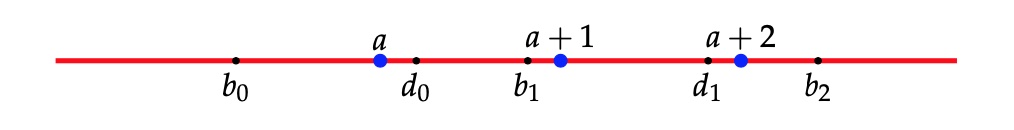and from the inequalities above this implies that both $(a+1,b+1)$ and $(a+1,b+2)$ are in $W$, giving a short interval $S$ in the projection.

(2) : If $\lceil (1+\tau^2)c + (b+1)\tau \rceil – \lceil (1+\tau^2)c+b \tau \rceil =1$, then there must be an integer $a$ such that $(1+\tau^2)c+b \tau < a < (1+\tau^2)cv + (b+1)\tau -1$, giving the situation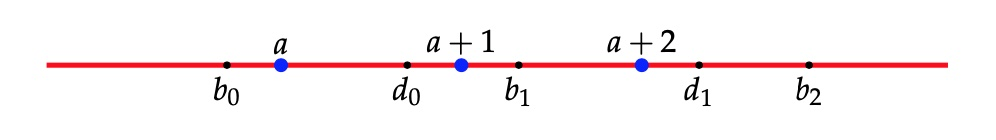giving from the inequalities that both $(a+1,b+1)$ and $(a+2,b+1)$ are in $W$, giving a long interval $L$ in the projection, finishing the proof.

Before we’ll come to applications of quasicrystals to viruses it is perhaps useful to illustrate essential topics such as deflation, inflation, aperiodicity, local isomorphism and the cut-and project method in the simplest of cases, that of $1$-dimensional tilings.

We want to tile the line $\mathbb{R}^1$ with two kinds of tiles, short ($S$) and ($L$) long intervals, differing by a golden ratio factor $\tau=\tfrac{1}{2}(1+\sqrt{5}) \approx 1.618$.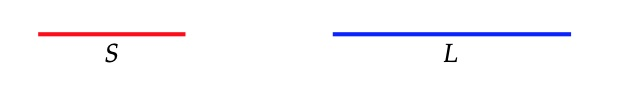Clearly, no two tiles may overlap and we impose a gluing restriction: there can be no two consecutive $S$-intervals in the tiling, and no three consecutive $L$-intervals.

The code of a tiling is a doubly infinite word in $S$ and $L$ such that there are no two consecutive $S$’s nor three consecutive $L$’s. For example
$\sigma = \dots LSLLSLS\underline{L}LSLLSL \dots$
We underline one tile to distinguish the sequence from shifts of it.

Conway’s musical sequences will be special codes (or tilings), allowing for the inverse operations of inflation and deflation, terms coined by John Conway in relation to Penrose tilings. The musical sequences are important to understand Conway’s worms (sometimes called “wormholes”) which are strings of Long and Short bow ties in a Penrose tiling, and to measure the distances between Amman bars. In fact, many of the properties of Penrose tilings and $3$-dimensional quasicrystals (for example, local isomorphism) have their counterparts for tilings having a musical sequence as code.Conway’s investigations of Penrose tiles held up work on the ATLAS-project and caused some problems at home:

“In pursuing his investigations, he unsurped some of his wife Eileen’s territory, covering the dining table with an infinite nuisance of tiles. He cut them out himself, causing his right hand to hurt with cramps for days. To Eileen’s dismay, he studied the dining table mosaic for a year, relegating family meals to the kitchen and prohibiting dinner parties.”

From “Genius at Play – The curious mind of John Horton Conway” by Siobhan Roberts

Let’s investigate inflation and deflation of these tilings.

The point of the golden factor $\tau$ is to allow for deflation. That is, we can replace a tiling by another one with tiles $S$ and $L$ both a factor $\tfrac{1}{\tau}=\tau-1 \approx 0.618$ smaller than the original tiles. If the original $S$-tile has length $a$ (and the $L$-tile length $\tau a$), then the new tile $S$ wil have length $\tfrac{1}{\tau}a$ and the new $L$-tile length $a$.
We do this by replacing each old $S$-tile by a new $L$-tile, and to break up any old $L$-tile in a new $L$ and new $S$-tile, as $\tau a = a + \tfrac{1}{\tau}a$ (note that $\tau^2=\tau+1$)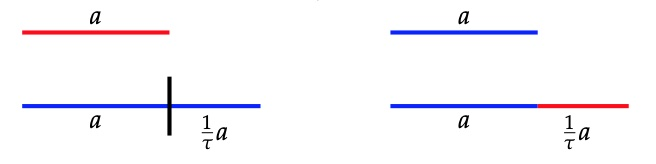To get the code of the deflated tiling we replace each letter $S$ by a letter $L$ and each $L$ by $LS$. The underlined letter will be the first letter of the deflated underlined letter in the original sequence. The deflated sequence of the one above is
$def(\sigma) = \dots LSLLSLSLLSL\underline{L}SLSLLSLSLLS \dots$
and it is easy to see that the deflated tiling satisfies again the gluing condition.

Certain of these tilings (not all!) allow for an inverse to deflation, called inflation, increasing the size of the tiles by a factor $\tau$.

Starting from a tiling we divide each $L$-tile in half and these mid-points will be end-points of the tiles in the new tiling, erasing all endpoints of the original one. The inflated tiling will have two sorts of tiles, a new short one $S$ of length $\tau a$ obtained from the end-half of an original $L$-tile, followed b the start-half of an original $L$-tile, and a new long tile $L$ of length $\tau^2 a = (\tau+1) a$, made of the end-half of an original $L$, followed by an original $S$, followed by the start-half of an original $L$.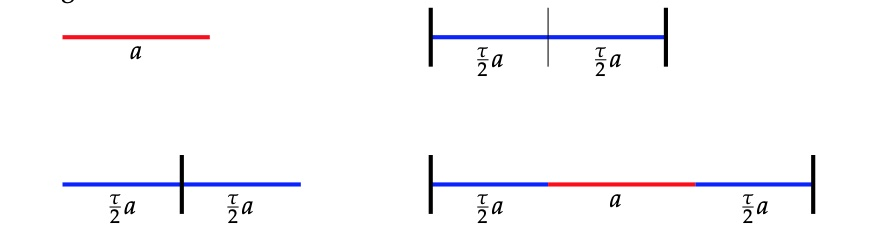We get the code of the inflated tiling by replacing first each $L$ by $ll$ and subsequently replace each word $lSl$ by a letter $L$ and each $ll$ by $S$. An example,
$\sigma = \dots LSLLSLSLLSL\underline{L}SLSLLSLSLLS \dots \\ \dots llSllllSllSllllSlll\underline{l}SllSllllSllSllllS \dots \\ inf(\sigma) = \dots (l)LSLLSLS\underline{L}LSLLS(lS) \dots$

But, the inflated tiling may no longer satisfy the gluing condition. An example
$\dots LSLSLSL \dots \mapsto \dots llSllSllSll \dots \mapsto \dots (l)LLL(l) \dots$

A Conway musical sequence is the code of a tiling $\sigma$ such that all its consecutive inflations $inf^n(\sigma)$ satisfy the gluing condition. For the corresponding Conway tiling $\sigma$ we have that
$def(inf(\sigma))=\sigma=inf(def(\sigma))$

Let’s construct at least two such Conway tilings (later we’ll see that there are uncountably many). Take $C_n=def^n(LS\underline{L})$ and write it in a special form to highlight symmetries.
\begin{eqnarray*}
C_0 =& (L.S)\underline{L} \\
C_1 =& L(S.L)\underline{L}S \\
C_2 =& LSL(L.S)\underline{L}SL \\
C_3 =& LSLLSL(S.L)\underline{L}SLLS \\
C_4 =& LSLLSLSLLSL(L.S)\underline{L}SLLSLSL
\end{eqnarray*}

The even terms have middle-part $(L.S)$ and the odd ones $(S.L)$. The remaing left and right parts are each others reflexion (or part of it). This is easily seen by induction as are the inclusions
$C_0 \subset C_2 \subset C_4 \subset \dots \subset C_{even} \quad \text{and} \quad C_1 \subset C_3 \subset C_5 \subset \dots \subset C_{odd}$

$C_{even}$ and $C_{odd}$ are special Conway musical sequences, called the middle $C$-sequences, and are each others inflation and deflation. If you are familiar with Penrose tilings, these are the $1$-dimensional counterparts of the cartwheel Penrose tiling (here with the $10$ Conway worms emanating from the center, and with the borders of the first few cartwheels drawn).A direct consequence of inflation on Conway’s musical sequences is that the corresponding tiling is aperiodic, that is, it has no translation symmetry.

For, inflation only depends on the local configuration of tiles, so if translation by $R$ is a symmetry of a musical sequence $\sigma$ then it is also a symmetry of $inf(\sigma)$, and so also of $inf^n(\sigma)$. But for large $n$ we will have that $R < \tau^n a$ (with $a$ the size of the tiles in $\sigma$). But then a tile in $inf^n(\sigma)$ and its translation by $R$ must overlap which is impossible if $+R$ is a translation symmetry of $inf^n(\sigma)$. Done!

Returning to the middle C-sequences, what was the point of starting with $C_0 = LSL$? Well, it follows directly from the gluing restrictions that any letter in a musical sequence is part of a subword $LSL$ of $\sigma$. But then, every finite subword $W$ of $\sigma$ is also a subword of $C_{2n}$ for some large $n$.

For, let $d$ be the length of the interval corresponding to $W$ and choose $n$ such that $d > \tau^{2n} a$ then the interval of the line corresponding to $W$ is contained in a single tile in $inf^{2n}(\sigma)$ and this tile belongs to a subword $LSL$ of $inf^{2n}(\sigma)$. But then $W$ will be a subword of the $2n$-th deflation of that interval $LSL \subset inf^{2n}(\sigma)$, which is $C_{2n}$.

Or, as Conway would phrase it with respect to Penrose tilings (quote again from Siobhan Roberts’ book)

“Every points is in the cartwheel somewhere. If you jab your finger anywhere, on any point anywhere on teh pattern, you are part of a cartwheel. The whole ting is overlapping cartwheels.”

An immediate consequence is the local isomorphism theorem: Every subword of a musical sequence $\sigma$ appears infinitely many times as subword of any other musical sequence. That is, one cannot distinguish two tilings of the line with musical sequence codes from each other by looking at finite intervals!

The argument is similar to the one above. The finite interval corresponding to the subword lies in a unique tile of $inf^n(\sigma)$ for $n$ large enough. Now, take another musical sequence $\mu$ and consider any of the infinitely many tiles of the same type in $inf^n(\mu)$, then $def^n$ of such a tile will contain the subword in $\phi$.

Another time, we’ll see that musical sequences can be produced by the ‘cut-and-project’-method (what I called the ‘windows’-method before).
This time we will project parts of the standard $2$-dimensional lattice $\mathbb{Z}^2$ onto the line, which is a lot easier to visualise than de Bruijn’s projection from $\mathbb{R}^5$ to produce Penrose tilings or the projection from six dimensional space to harvest quasicrystals.

If you look around for mathematical theories of the structure of viruses, you quickly end up with the work of Raidun Twarock and her group at the University of York.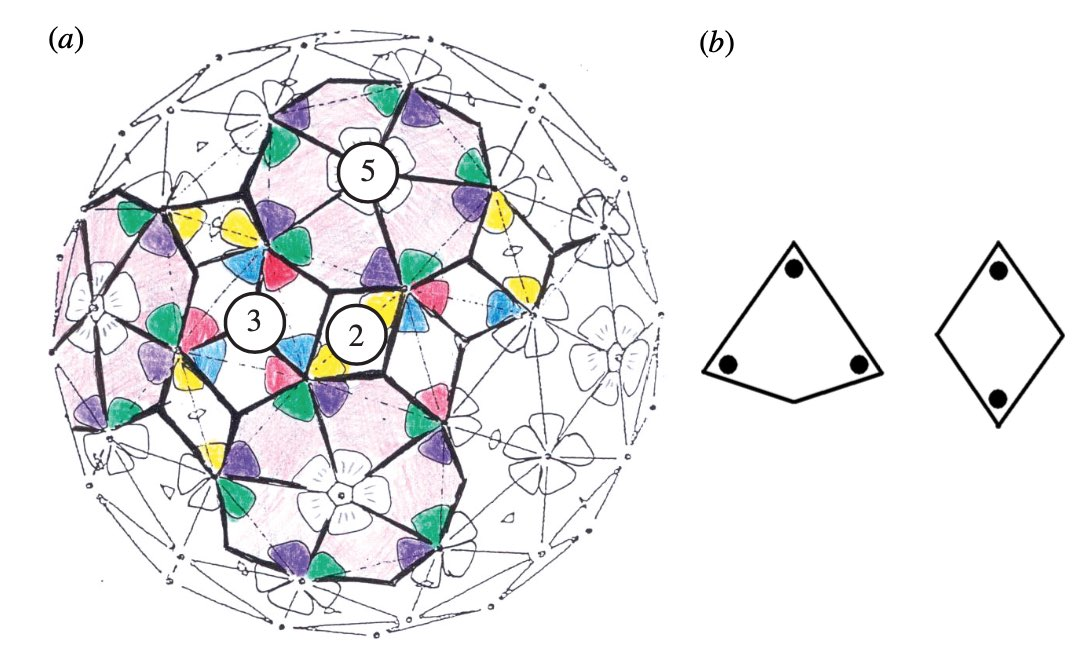We’ve seen her proposal to extend the Caspar-Klug classification of viruses. Her novel idea to distribute proteins on the viral capsid along Penrose-like tilings shouldn’t be taken too literally. The inherent aperiodic nature of Penrose tiles doesn’t go together well with perfect tilings of the sphere.

Instead, the observation that these capsid tilings resemble somewhat Penrose tilings is a side-effect of another great idea of the York group. Recently, they borrowed techniques from the theory of quasicrystals to gain insight in the inner structure of viruses, in particular on the interaction of the capsid with the genome.

By the crystallographic restriction theorem no $3$-dimensional lattice can have icosahedral symmetry. But, we can construct aperiodic structures (quasicrystals) which have local icosahedral structure, much like Penrose tilings have local $D_5$-symmetry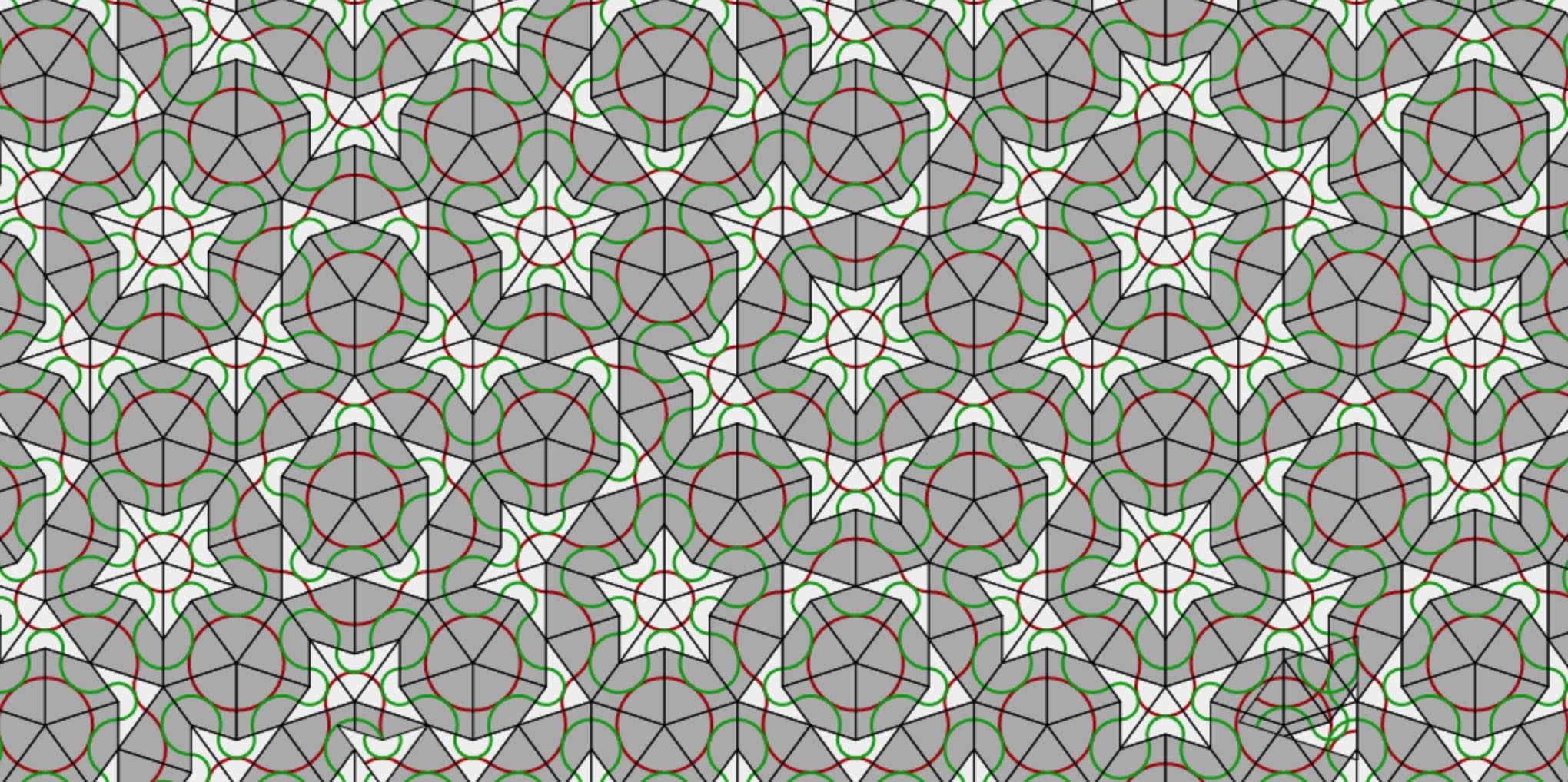This is best explained by de Bruijn‘s theory of pentagrids (more on that another time). Here I’ll just mention the representation-theoretic idea.

The isometry group of the standard $5$-dimensional lattice $\mathbb{Z}^5$ is the group of all signed permutation $5 \times 5$ matrices $B_5$ (Young’s hyperoctahedral group). There are two distinct conjugacy classes of subgroups in $B_5$ isomorphic to $D_5$, one such subgroup generated by the permutation matrices
$x= \begin{bmatrix} 0 & 1 & 0 & 0 & 0 \\ 0 & 0 & 1 & 0 & 0 \\ 0 & 0 & 0 & 1 & 0 \\ 0 & 0 & 0 & 0 & 1 \\ 1 & 0 & 0 & 0 & 0 \end{bmatrix} \qquad \text{and} \qquad y = \begin{bmatrix} 1 & 0 & 0 & 0 & 0 \\ 0 & 0 & 0 & 0 & 1 \\ 0 & 0 & 0 & 1 & 0 \\ 0 & 0 & 1 & 0 & 0 \\ 0 & 1 & 0 & 0 & 0 \end{bmatrix}$
The traces of $x,x^2$ and $y$, together with the character table of $D_5$ tell us that this $5$-dimensional $D_5$-representation splits as the direct sum of the trivial representation and of the two irreducible $2$-dimensional representations.
$\mathbb{R}^5 = A \simeq T \oplus W_1 \oplus W_2$
with $T = \mathbb{R} d$, $W_1 = \mathbb{R} u_1 + \mathbb{R} u_2$ and $W_2 = \mathbb{R} w_1 + \mathbb{R} w_2$ where
$\begin{cases} (1,1,1,1,1)=d \\ (1,c_1,c_2,c_3,c_4)= u_1 \\ (0,s_1,s_2,s_3,s_4) = u_2 \\ (1,c_2,c_4,c1,c3)= w_1 \\ (0,s_2,s_4,s_1,s_3)= w_2 \end{cases}$
and $c_j=cos(2\pi j/5)$ and $s_j=sin(2 \pi/5)$. We have a $D_5$-projection
$\pi : A \rightarrow W_1 \quad (y_0,\dots,y_4) \mapsto \sum_{i=0}^4 y_i(c_i u_1+s_i u_2)$
The projection maps the vertices of the $5$-dimensional hypercube to a planar configuration with $D_5$-symmetry.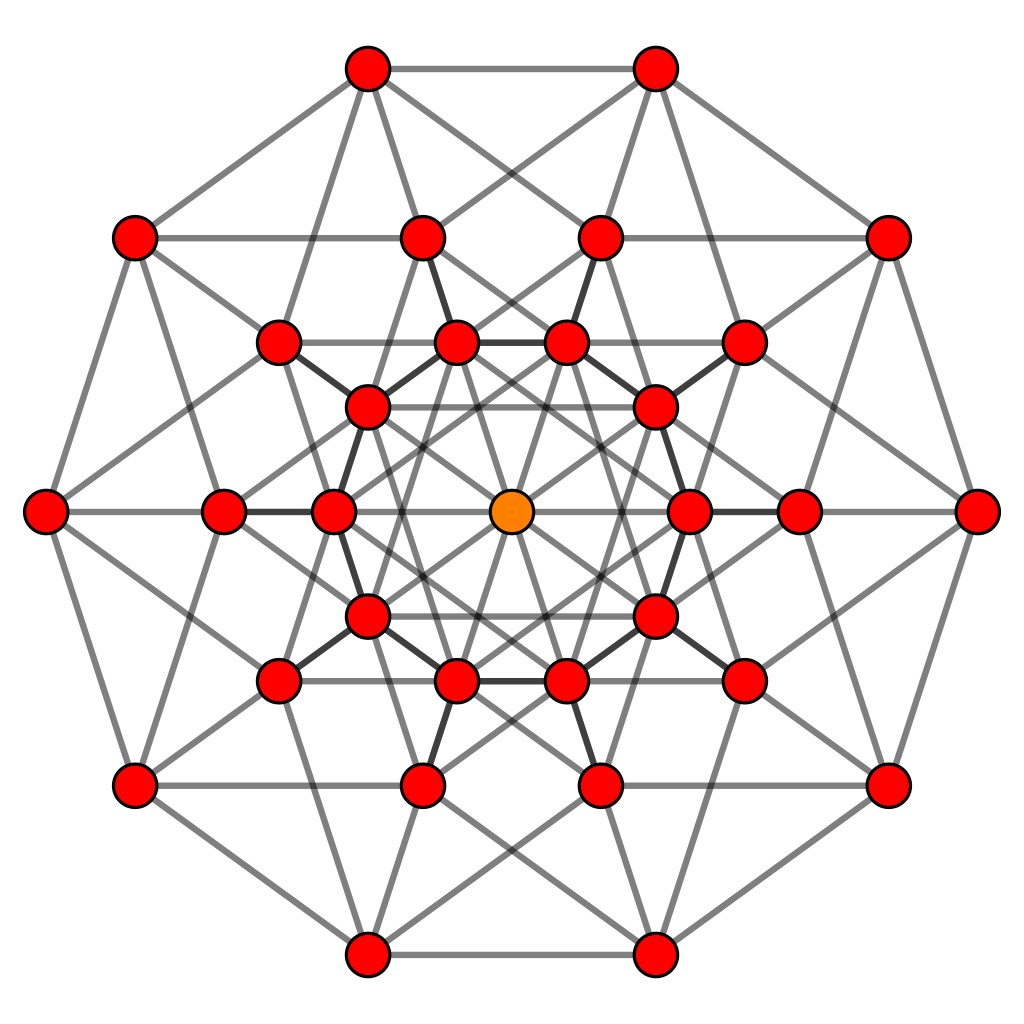de Bruijn’s results say that if we take suitable ‘windows’ of lattice-points in $\mathbb{Z}^5$ and project them via the $D_5$-equivariant map $\pi$ onto the plane, then the images of these lattice points become the vertices of a rhombic Penrose tiling (and we get all such tilings by choosing our window carefully).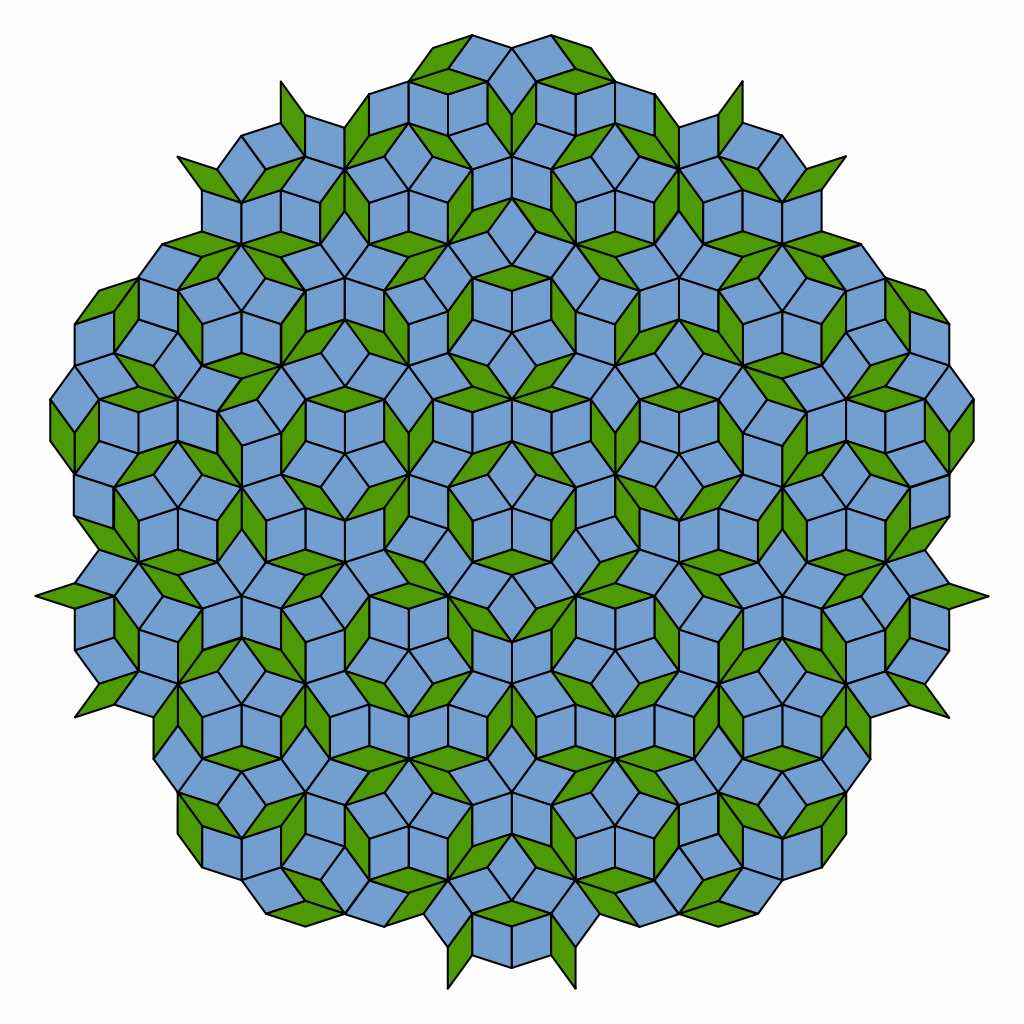This explains why Penrose tilings have a local $D_5$-symmetry. I’ll try to come back to de Bruijn’s papers in future posts.

But, let’s go back to viruses and the work of Twarock’s group using methods from quasicrystals. Such aperiodic structures with a local icosahedral symmetry can be constructed along similar lines. This time one starts with the standard $6$-dimensional lattice $\mathbb{Z^6}$ with isometry group $B_6$ (signed $6 \times 6$ permutation matrices).

This group has three conjugacy classes of subgroups isomorphic to $A_5$, but for only one of them this $6$-dimensional representation decomposes as the direct sum of the two irreducible $3$-dimensional representations of $A_5$ (the decompositions in the two other cases contain an irreducible of dimension $4$ or $5$ together with trivial factor(s)). A representant of the crystallographic relevant case is given by the signed permutation matrices
$x= \begin{bmatrix} 0 & 1 & 0 & 0 & 0 & 0 \\ 1 & 0 & 0 & 0 & 0 & 0 \\ 0 & 0 & 0 & -1 & 0 & 0 \\ 0 & 0 & -1 & 0 & 0 & 0 \\ 0 & 0 & 0 & 0 & -1 & 0 \\ 0 & 0 & 0 & 0 & 0 & -1 \end{bmatrix} \qquad \text{and} \qquad y= \begin{bmatrix} 0 & 0 & 1 & 0 & 0 & 0 \\ 1 & 0 & 0 & 0 & 0 & 0 \\ 0 & 1 & 0 & 0 & 0 & 0 \\ 0 & 0 & 0 & 0 & -1 & 0 \\ 0 & 0 & 0 & 0 & 0 & 1 \\ 0 & 0 & 0 & -1 & 0 & 0 \end{bmatrix}$

Again, using suitable windows of $\mathbb{Z}^6$-lattice points and using the $A_5$-equivariant projection to one of the two $3$-dimensional components, one obtains quasicrystals with local $A_5$-symmetry.

In this $3$-dimensional case the replacements of the thick and thin rhombi are these four parallellepipeda, known as the Amman blocks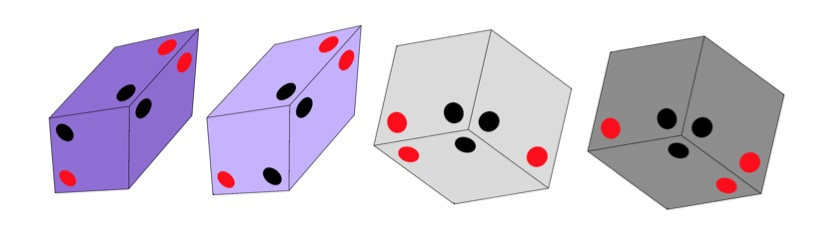which must be stacked together obeying the gluing condition that dots of the same colour must be adjacent.

Has anyone looked at a possible connection between the four Amman blocks (which come in pairs) and the four (paired) nucleotides in DNA? Just an idle thought…

These blocks grow into quasicrystals with local icosahedral symmetry.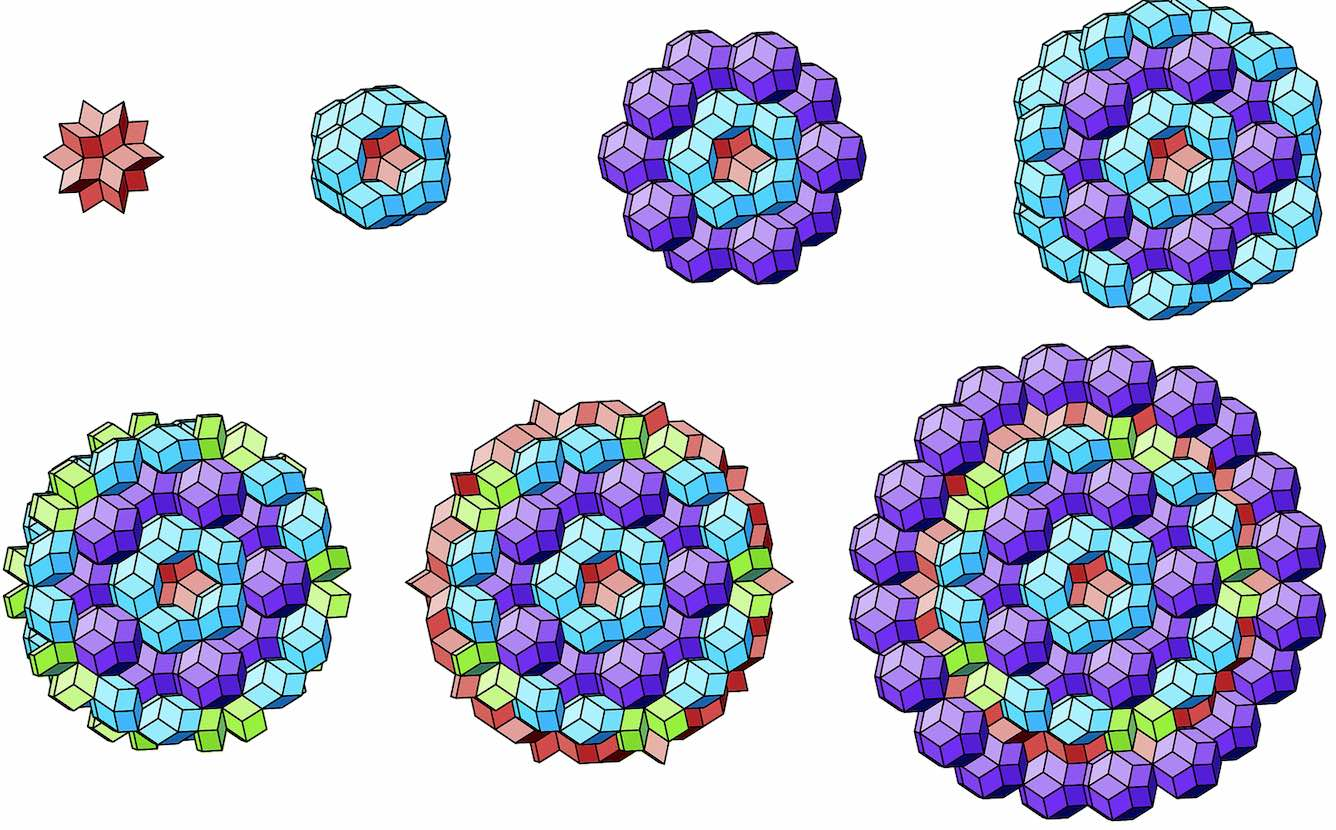The faces on the boundary of such a sphere-like quasicrystal then look a lot like a Penrose tiling.

How can we connect these group and representation-theoretic ideas to the structure of viruses? Here’s another thought-provoking proposal coming from the York group.

Take the $A_5$ subgroup of the hyperoctahedral group in six dimensiona $B_6$ generated by the above two matrices (giving a good $A_5$-equivariant projection $\pi$ to three dimensional space) and consider an intermediate group
$A_5 \subsetneq G \subseteq B_6$
Take a point in $\mathbb{R}^6$ and look at its orbit under the isometries of $G$, then all these points have the same distance from the origin in $\mathbb{R}^6$. Now, project this orbit under $\pi$ to get a collection of points in $\mathbb{R}^3$.

As $\pi$ is only $A_5$-equivariant (and not $G$-equivariant) the image points may lie in different shells from the origin. We can try to relate these shells of points to observational data on the inner structures of viruses.

Here’s a pretty convincing instance of such a correlation, taken from the thesis by Emilio Zappa “New group theoretical methods for applications in virology and quasicrystals”.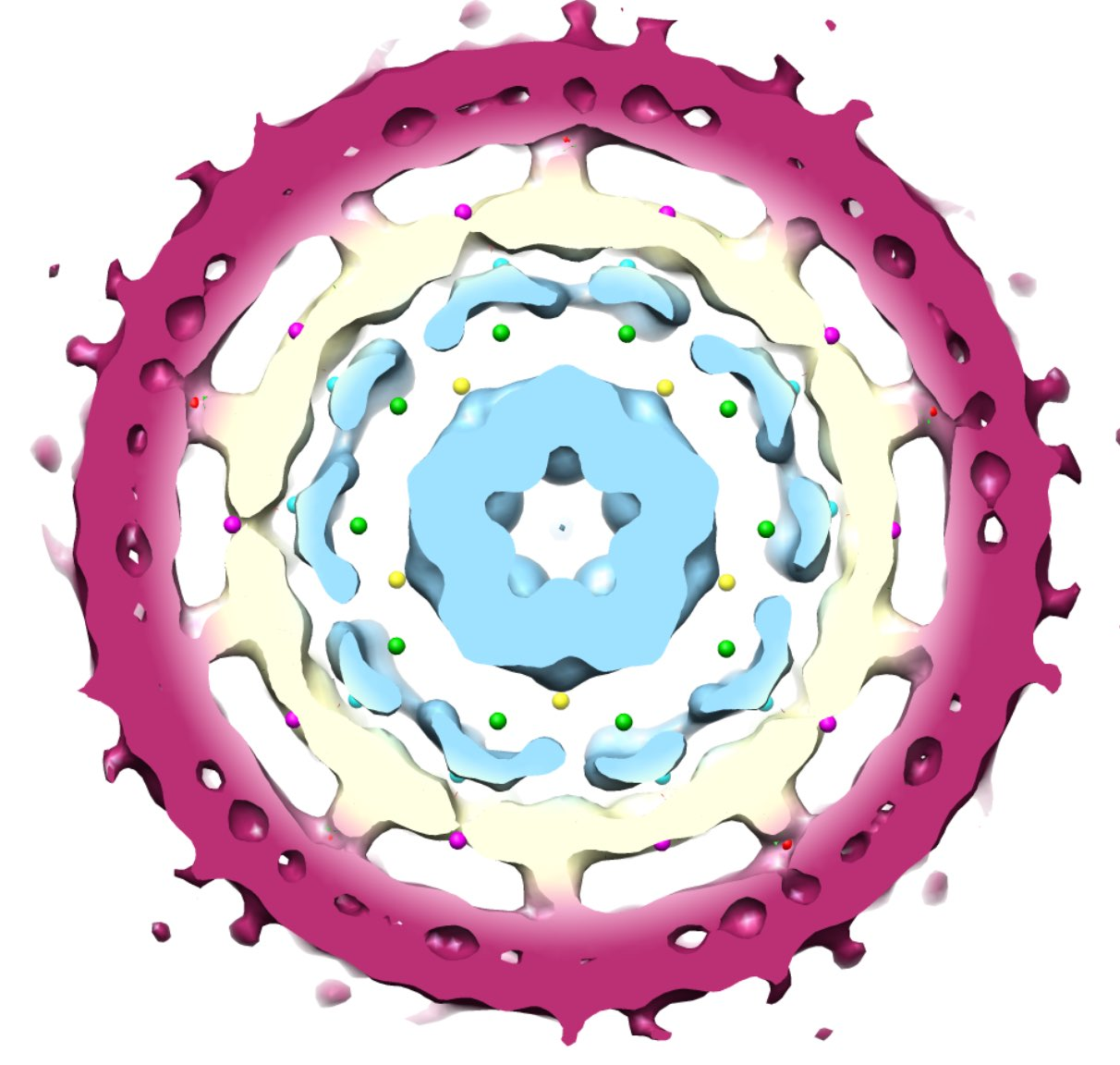This is the inner structure of the Hepatitis B virus, showing the envelope (purple), capsid protein (cream) and genome (light blue). The coloured dots are the image points in the different shells around the origin.

Do viruses invade us from the sixth dimension??

As you may have guessed from the symmetries of Covid-19 post, I did spend some time lately catching up with the literature on the geometric structure and symmetries of viruses. It may be fun to run a little series on this.

A virus is a parasite, so it cannot reproduce on its own and needs to invade a host cell to replicate. All information needed for this replication process is stored in a fragile DNA or RNA string, the viral genome.

This genome needs to be protected by a coating made of proteins, the viral capsid. Most viruses have an additional fatty protection layer, the envelope, decorated by virus (glyco)proteins (such as the ‘spikes’ needed to infiltrate the host cell).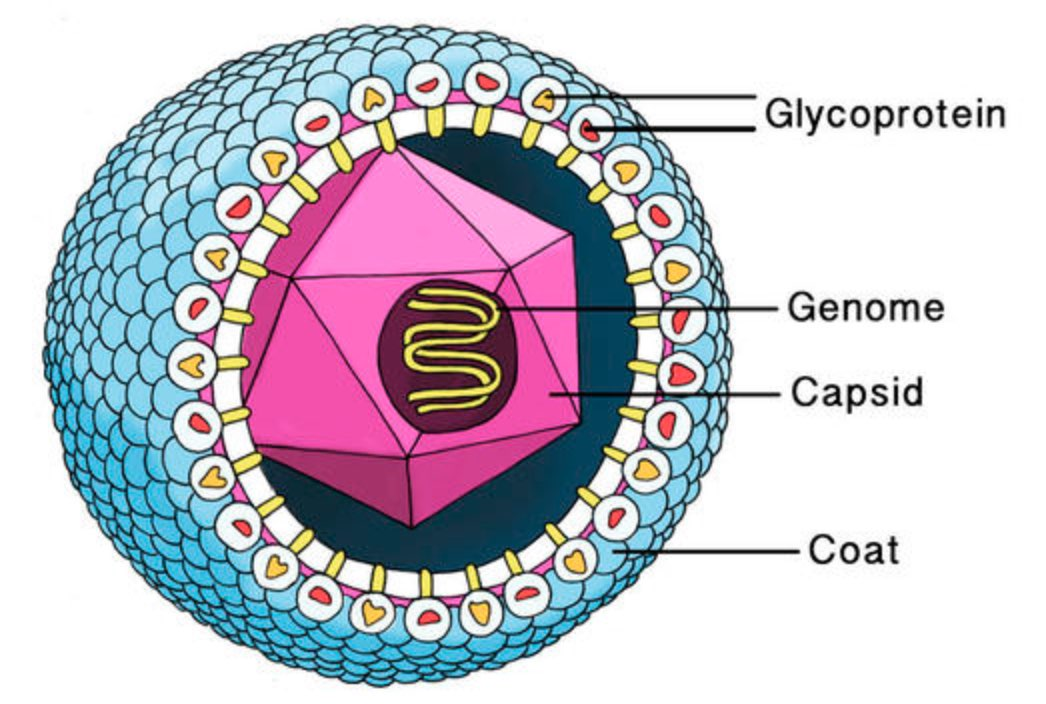Most viruses are extremely small (between 20 and 200nm), our friend the corona-virus measures between 80 and 120nm. So, its genome is also pretty small (the corona genome has around 30.000 base pairs). To maximise its information, the volume of the protective capsid must be as large as possible, and must be formed by just a few different proteins (to free as much space in the code of the genome for other operations) and clusters of them are distributed over the polyhedral capsid, as symmetric as possible.

This insight led Watson and Crick, the discoverers of the structure of DNA, to the ‘genetic economy’-proposal that most sphere-like viruses will have an icosahedral capsid because the icosahedron is the Platonic solid with the largest volume and rotational symmetry group. They argued that the capsid is most likely constructed from a single subunit (capsomere), which is repeated many times to form the protein shell.Little is known about capsid formation, that is the process in which the capsid proteins self-assemble into an icosahedral shape, nor about the precise interplay between the genome and the capsid proteins. If we would understand these two things better it might open new possibilities for anti-viral drugs, by either blocking the self-assembly process or by breaking the genome-capsid interaction.

A first proposal for the capsid structure was put forward by Caspar and Klug. Their quasi-equivalence principle asserts that each of the 20 triangular faces of the icosahedron is subdivided in 3 subunits, each consisting of at least one protein.

Most viruses have much more than 60 proteins in their capsid, so Caspar and Klug introduced their $T$-number giving the number of proteins per subunit. One superimposes the triangulation of the icosahedron with the hexagonal plane lattice, then $T$ is the number of sub-triangles of these hexagons contained in each subunit. For $T = 7$ we have the following situation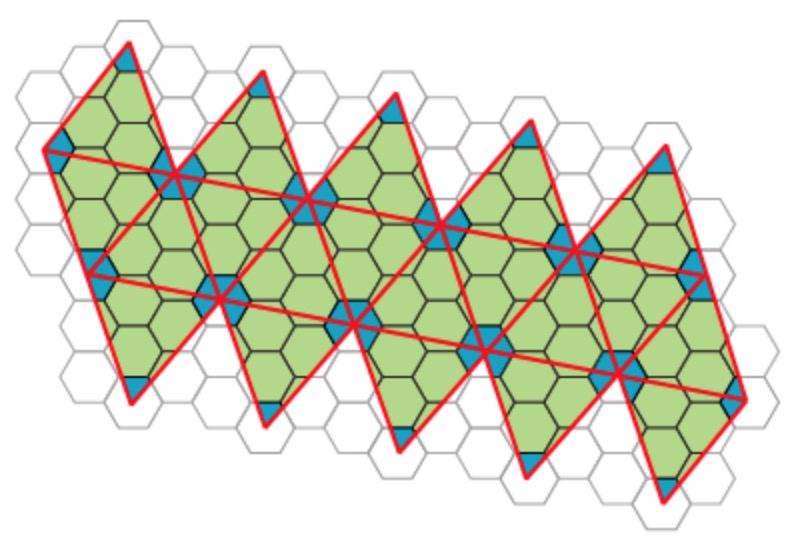Folding back the triangulation to form the icosahedron one then obtains a tiling consisting of hexagons (the green regions) and pentagons (the blue regions)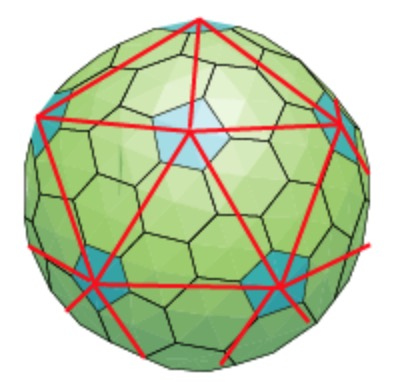It turned out that many viruses with icosahedral symmetry consist of subunits having a different number of proteins, such as dimers (2 proteins), trimers (3 proteins), or pentamers (5 proteins) and these self-organise around a 2, 3, or 5-fold rotational axis of the icosahedron.

This led Reidun Twarock around 2000 to propose her virus tiling theory. This is a generalisation of the Caspar-Klug theory in which one superimposese the triangulation of the icosahedron with other tilings of the plane, consisting of two or more non-congruent tiles. Here an example which looks a bit like the aperiodic Penrose tilings of the plane.Here’s a recent Quanta-Magazine article on Twarock’s work and potential consequences: The illuminating geometry of viruses.

And here’s an LMS Popular Lecture, from 2008, by Raidun Twarock herself: “Know your enemy – viruses under the mathematical microscope”.

A natural question these days might be: “what are the rotational symmetries of the Covid-19 virus?”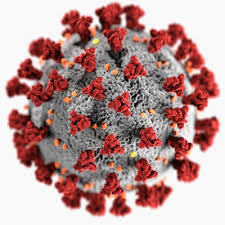Most illustrations show a highly symmetric object, suggesting it might have icosahedral symmetry. In fact, many viruses do have icosahedral symmetry as a result of the ‘genetic economy principle’ proposed by Watson and Crick in 1956, resulting in the Caspar-Klug classification of viral capsids.

But then, perhaps this icosahedral illusion is a result of design decisions illustrators made turning scientific data into pictures. Veronica Falconieri Hays wrote a beautiful article describing the effort going into this: How I built a 3d-model of the coronavirus for Scientific American. Here’s her final picture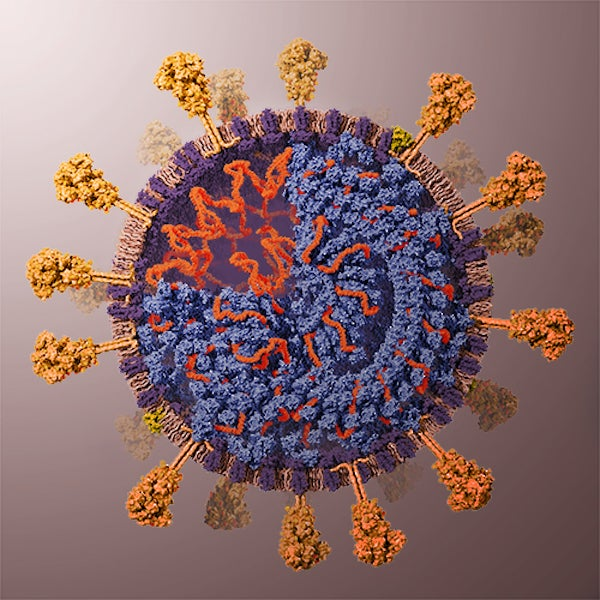And yes, icosahedral symmetry was one of her design decisions:

The M proteins form pairs, and it is estimated that there are 16–25 M proteins per spike on the surface of the virus. I ended up modeling 10 M protein pairs (so 20 M proteins) per spike in my model. Some researchers hypothesize that the M proteins form a lattice within the envelope (interacting with an underlying lattice of N proteins; see below). I decided to use an icosahedral sphere to create a regular distribution of the M protein dimers to hint at this hypothesis.

The spikes (or S-proteins) are the tentacles in these pictures, and one of the few hard figures on Corona is that ‘on average’ there are 74 of them.

This fact is enough to rule out icosahedral symmetry.

If the icosahedral rotation group (of order $60$, isomorphic to $A_5$) acts on the $74$ spikes, then each orbit consists of $60$ spikes unless that spike lies on a twofold, threefold or fivefold rotation axis, in which cases the number of spikes in its orbit are respectively $30$, $20$ or $12$. So, we can’t get a total number of $74$ spikes!

However, just looking at the number of spikes we cannot rule out octahedral symmetry!

The octahedral rotation group (of order $24$, isomorphic to $S_4$) will have orbits of size $24$ unless the spike lies on a twofold, threefold or fourfold rotation axis, giving orbits of size $12$, $8$ and $6$ respectively (the midpoints of edges, the vertices and the midpoints of faces of the octahedron), and

$74 = 24+24+12+8+6$

The most symmetric arrangement of spikes would be to subdivide each of the $8$ triangular faces of the octahedron into $6$ triangles with vertices the midpoint of the face, a vertex and a midpoint of an edge, and then to position the spikes on the axis through the vertices and midpoints of these smaller triangles.

Googling around I found very few references to symmetries of Covid-19, probably because it has an helical RNA-coil, which seems not to go well with Caspar-Klug type polyhedral viral capsids.

Here’s an exception: A structural model for the Coronavirus nucleocapsid by Federico Coscio, Alejandro D. Nadra, and Diego U. Ferreiro.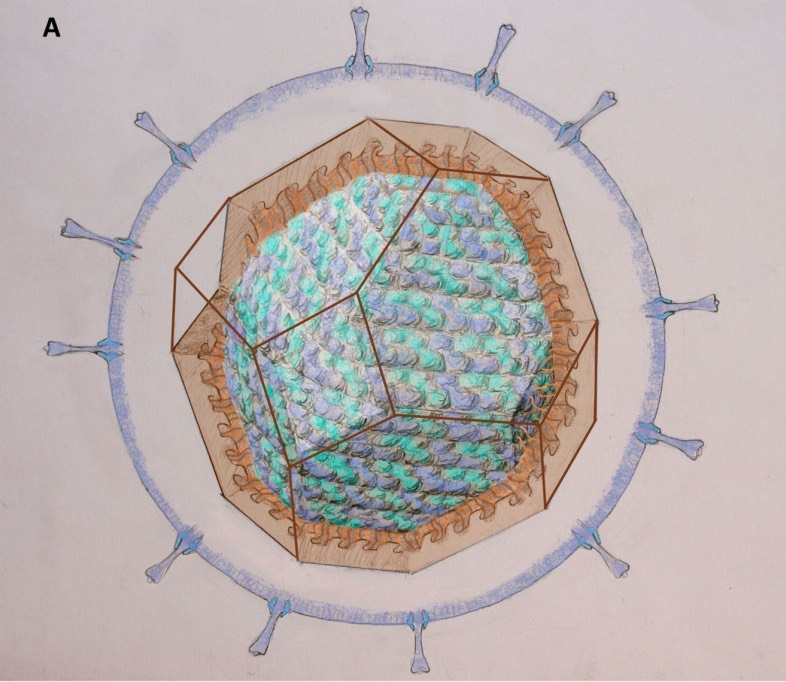They propose a truncated octahedron as capsid (in transparent brown) with interior a continuous coil packing of blue and cyan helices. The virus membrane with the spikes and M proteins is drawn in blue.

If you have better info or references on the (conjectural) symmetries of Covid-19, please leave a comment.